Home   »   Important Question   »   Important Questions for Class 12 Computer...

# Important Questions for Class 12 Computer Science Term 2 with Answers

## Important Questions for Class 12 Computer Science Term 2

Important Questions for Class 12 Computer Science Term 2: The Central Board of Secondary Education will conduct the CBSE Class 12th Term 2 Computer Science exam on 13th June 2022. The students appearing in the Class 12th Term 2 Computer Science exam can practice the Important Questions for Class 12 Computer Science Term 2 to get good marks in computer science. The Important Questions for Class 12 Computer Science Term 2 is prepared by the expert faculties of Adda247, the students can practice Important Questions for Class 12 Computer Science Term 2 to assess their preparation for the Term 2 examination. Go through the all of the Important Questions for Class 12 Computer Science Term 2 and bookmark this page to get CBSE Term 2 Computer Science Answer Key 2022.

## Important Questions for Class 12 Computer Application Term 2 with Answers: Computational Thinking and Programming

Question 1:
Write the definition of a function AddUp(int Arr[ ], int N) in C++, in which all even positions (i.e. 0,2,4 ) of the array should be added with the content of the element in the next position and odd positions (i.e. 1,3,5, ) elements should be incremented by 10. All India 2017
NOTE

• The function should only alter the content in the same array.
• The function should not copy the altered content in another array.
• The function should not display the altered content of the array.
• Assuming, the Number of elements in the array are Even.

Аnswer:

void AddUp(int Arr[], int N)
{
for(int i=0;i<N;i++)
{
if(i%2==0)
Arr[i] += Arr[i+1];
else
Arr[i] += 10;
}
}

Question 2:
Write the definition of a function FixPay(float Pay[ ], int N) in C++, which should modify each element of the array Pay having N elements, as per the following rules:
Аnswer:

void FixPay(float Pay[], int N)
{
for(int i=0;i<=N—1;i++)
{
if(Pay[i]<100000)
Pay[i] = Pay[i]+(Pay[i]*25)/100;
else if(Pay[i]>=100000 && Pay[i]<200000)
Pay[i] = Pay[i]+(Pay[i]*20)/100;
else
Pay[i] = Pay[i]+(Pay[i]*15)/100;
}
}

Question 3:
Write the definition of a function FixSalary(float Salary[ ], int N) in C++, which should modify each element of the array Salary having N elements, as per the following rules:
Аnswer:

void FixSalary(float Salary[], int N)
{
for(int i=0;i<=N—1;i++)
{
if(Salary[i]<100000)
Salary[i]=Salary[i]+(Salary[i]*35)/100;
else if(Salary[i]>=100000 && Salary[i]<200000)
Salary[i]=Salary[i]+(Salary[i]*30)/100;
else
Salary[i]=Salary[i]+(Salary[i]*20)/100;
}
}

Question 4:
Write the definition of a function Alter (int A[ ], int N) in C++, which should change all the multiples of 5 in the array to 5 and rest of the elements as 0. e.g. if an array of 10 integers is as follows:
Аnswer:

void Alter(int A[], int N)
{
for(int i=0;i<10;i++)
{
if(A[i]%5==0)
A[i]=5;
else
A[i]=0;
}
}

Question 5:
Write the definition of a function Changeant P[ ], int N) in C++, which should change all the multiples of 10 in the array to 10 and rest of the elements as 1.  All India 2015
Аnswer:

void Change(int P[], int N)
{
for (int i=0;i<N;i++)
{
if (P[i]%10 == 0)
P[i] = 10;
else
P[i] = 1;
}
}

Question 6:
Write the definition of a function Modify(int A[ ], int N) in C++, which should reposition the content after swapping each adjacent pair of numbers in it.
[NOTE Assuming the size of array is multiple of 4]
Аnswer:

void Modify(int A[], int N)
{
for(int i=0;i<N;i++)
{
int temp = A[i];
A[i] = A[i+2];
A[i+2] = temp;
if(i%4>=1)
i = i+2;
}
}

Question 7:
Write code for a function void oddEven(int S[ ], int N) in C++, to add 5 in all the odd values and 10 in all the even values of the array S.
Аnswer:

void oddEven(int S[],int N)
{
for(int i=0;i<N;i++)
{
if(S[i]%2 == 0)
S[i] += 10;
else
S[i]+=5;
}
}

Question 8:
Write code for a function void EvenOdd (int T [ ], int C) in C++, to add 1 in all the odd values and 2 in all the even values of the array T.
Аnswer:

void EvenOdd(int T[],int C)
{
for(int i=0;i<C;i++)
{
if(T[i]%2 == 0)
T[i] += 2;
else
T[i] += 1;
}
}

Question 9:
Write a function in C++ TWOTOONE( ) which accepts two array X[ ], Y[ ] and their size n as argument. Both the arrays X[ ] and Y[ ] have the same number of elements. Transfer the content from two arrays X[ ], Y[ ] to array Z[ ]. The even places (0,2,4….) of array Z[ ] should get the contents from the array X[ ] and odd places (1,3,5…) of array Z[ ] should get the contents from the array Y[ ].
Example: If the X[ ] array contains 30,60,90 and the Y[ ] array contains
10.20.50. Then Z[ ] should contain
30.10.60.20.90.50. All India 2014
Аnswer:

void TW0T00NE(int X[], int Y[], int n)
{
int Z, i,j=0,k=0;
for(i=0;i<(n+n);i++)
{
if(i%2 == 0)
{
Z[i] = X[j];
j++;
}
else
{
Z[i] = Y[k];
k++;
}
}
}

Question 10:
Write code for a function void Convert (int T[ ], int Num) in C++, which repositions all the elements of the array by shifting each of them one to one position before and by shifting the first element to the last position.
Аnswer:

void Convert(int T[], int Num)
{
int temp = T;
for(int i=0;i<(Num-1);i++)
{
T[i]=T[i+l];
}
T[i]= temp;
}

Question 11:
Write code for a function void ChangeOver(int P[ ], int N) in C++, which re-positions all the elements of the array by shifting each of them to the next position and by shifting the last element to the first position.
Аnswer:

void ChangeOver(int P[],int N)
{
int temp;
for(int i=0;i<(N-1);i++)
{
temp = P[N-1];
P[N-1] = P[i];
P[i] = temp;
}
}

Question 12:
Write the definition for a function void Transfer (int A, int B) in C++, which takes two integer arrays, each containing 6 elements as parameters. The function should exchange all odd places (i.e. 3rd and 5th) of the two arrays.
Аnswer:

void Transfer(int A,int B)
{
int i,temp;
for(i=1;i<=5;i=i+2)
{
temp = A[i];
A[i] = B[i];
B[i] = temp;
}
}

Question 13:
Write a function SWAP2CHANGE(int p[ ], int N) in C++ to modify the content of the array in such a way that the elements, which are multiples of 10 swap with the value present in the very next position in the array.
e.g. If the content of array p is
91, 50, 54, 22, 30, 54
The content of array p should become
91, 54, 50, 22, 54, 30
Аnswer:

void SWAP2CHANGE(int p[],int N)
{
int C,i;
for(i=0;i<=N-2;i++)
{
if(p[i]%10 == 0)
{
C = p[i];
p[i] = p[i+1];
p[i+1] = C;
i++:
}
}
}

Question 14:
Write a function SWAP2BEST(int ARR[ ], int Size) in C++ to modify the content of the array in such a way that the elements, which are multiples of 10 swap with the value present in the very next position in the array.
e.g. If the contents of array ARR are
90, 56, 45, 20, 34, 54
The contents of array should become
56, 90, 45, 34, 20, 54  All India 2012
Аnswer:

void SWAP2BEST(int ARR[], int Size)
{
int i,C;
for(i=0;i<=Size-2;i++)
{
if(ARR[i]%10==0)
{
C=ARR[i];
ARR[i] = ARR[i+1];
ARR[i+1] = C;
i++;
}
}
}

Question 15:
Write a Get2From1( ) function in C++ to transfer the content from one array ALL[ ] to two arrays Odd[ ] and Even[ ]. The Even[ ] array should contain the values from places(0, 2, 4, …) of array ALL[ ] and Odd[ ] array should contain the values from odd position like (1, 3, 5, …).
e.g. The ALL[ ] array should contain 30,10, 60, 50, 90, 80
If the Even[ ] array contains 30, 60, 90
and the Odd[ ] array contains
10, 50, 80 All India 2011
Аnswer:

void Get2From1(int ALL[], int N)
{
int p,q,i,j=0,k=0;
if(N%2 == 0)
{
p = N/2;
q = N/2;
}
else
{
p = N/2;
q = ((N/2)+l);
}
int *0dd = new int[p];
int *Even = new int[q];
for(i=0;i<(N);i++)
{
if(i%2 == 0)
Even[j++]=ALL[i];
else
Odd[k++]=ALL[i];
}
}

Question 16:
Write a function CHANGE( ) in C++, which accepts an array of integer and its size as parameters and divide all those array elements by 7, which are divisible by 7 and multiply other array elements by 3.
Аnswer:

void CHANGE(int A[],int N)
{
for(int i=0;i<N;i++)
if(A[i]%7 == 0)
A[i]=A[i]/7;
else
}
A[i] = A[i]*3;
}
}

Question 17:
Write a function REASSIGN( ) in C++, which accepts an array of integer and its size as parameters and divide all those array elements by 5, which are divisible by 5 and multiply other array elements by 2.
Аnswer:

void REASSIGN(int A[],int N)
{
for(int i=0;i<N;i++)
{
1f(A[i]%5 == 0)
A[i] = A[i]/5;
else
A[i] = A[i]*2;
}
}

Question 18:
Write a function SORTPOINTS( ) in C++ to sort an array of structure Game in descending order points using bubble sort.
NOTE Assume the following definition of structure Game.

struct Game
{
long PNo; //Player Number
char PName;
long Points;
}

Аnswer:

void SORTPOINTS(struct Game G[], int n)
{
int i, j;
struct Game t;
for(i=1;i<n;i++)
{
for(j=0;j<=n-i-1;j++)
{
if(G[j+1].Points>G[j].Points)
{
t = G[j];
G[j] = G[j+1]:
G[j+1] = t;
}
}
}
}

Question 19:
Write a function SORTSCORE( ) in C++to sort an array of structure. Examinee in descending order of Score using bubble sort.
NOTE Assume the following definition of structure Examinee.

struct Examinee
{
long Roll No;
char Name;
float Score;
};

Аnswer:

void SORTSCORE(struct Examinee ex[],int n)
{
int i,j;
struct Examinee t;
for(i=1;i<n;i++)
{
for(j=0;j<=n-i-1;j++)
{
if(ex[j+1].Score>ex[j].Score)
{
t = ex[j];
ex[j] = ex[j+1];
ex[j+1] = t;
}
}
}
}

Question 1:
Write a definition for a function SUMMIDCOLfint MATRIX[ ], int N, int M) in C++, which finds the sum of the middle column’s elements of the MATRIX (Assuming N represents number of rows and M represents number of columns, which is an odd integer). All India 2017
Example: If the content of array MATRIX having N as 5 and M as 3 is as follows:
The function should calculate the sum and display the following:
Sum of Middle Column : 15
Аnswer:

void SUMMIDCOL(int MATRIX[],int N,int M)
{
int j, SUM=0;
j=M/2;
for(int i=0;i<N;i++)
SUM += MATRIX[i][j];
cout<<"SUM of Middle Column:"<<SUM;
}

Question 2:
ARR is a two-dimensional array, which is stored in the memory along the row with each of its elements occupying 4 bytes. Find the address of the element ARR, if the element ARR is stored at the memory location 35000.
All India 2017
Аnswer:

B = 35000, W = 4 bytes, R=15, C=20, Lr=10, Lc=5, I=5, J=15
For row-wise allocation,
ARR = 35000+4[20(5-10)+(15-5)]
= 35000+4[20(-5)+10]
= 35000+4[-100+10]
= 35000+4[-90]
= 35000-360
= 34640

Question 3:
Write definition for a function DISPMID (int A[ ] , int R, int C) in C++ to display the elements of middle row and middle column from two dimensional array A having R number of rows and C number of columns.
Аnswer:

void DISPMID(int A[], int R, int C)
{
int i;
i=R/2;
for(int j=0;j<C;j++)
cout<<A[i][j]<<'\t';
count<<endl;
i=C/2;
for(j=0;j<R;j++)
cout<<A[j][i]<<'\t';
}

Question 4:
R is a two dimensional array, which is stored in the memory along the row with each of its element occupying 8 bytes, find the address of the element R, if the element R  is stored at the memory location 45,000. All India 2016
Аnswer:

B = 45000, R = 10, C = 50. W = 8 bytes, Lr = 8, Lc = 10, I = 5, J = 15
For row-wise allocation,
R[I][J] = B + W[C(I-Lr)+(J-Lc)]
R = 45000 + 8[50(5-8)+(15-10)]
= 45000 + 8[50x(-3)+5]
= 45000 + 8[-150+5]
= 45000 - 1160
= 43840

Question 5:
Write definition for a function SHOWMID(int P[ ], int R, int C) in C++ to display the elements of middle row and middle column from a two dimensional array P having R number of rows and C number of columns, e.g. if the content of array is as follows:
The function should display the following as output:
103 101 121 102 101
116 121 109 Delhi 2016
Аnswer:

void SHOWMID(int P[], int R, int C)
{
int i,j;
i=R/2;
for(j=0;j<C;j++)
cout<<P[i][j]<<'\t';
Cout<<endl;
i=C/2;
for(j-0;j<R;j++)
cout<<P[j][i]<<'\t';
}

Question 6:
T  is a two dimensional array, which is stored in the memory along the row with each of its element occupying 4 bytes, find the address of the element T, if the element T is stored at the memory location 52000. Delhi 2016
Аnswer:

B = 52000, R = 20, Ir= 10, C = 50 Ic = 8, W = 4bytes, I = 15, J = 5
For row-wise allocation,
T[I][J] = B+W[C(I-Lr)+(J-Lc)]
T = 52000 + 4[50(15-10)+(5-8)]
= 52000 + 4[50x5+(-3)]
= 52000 + 4[250-3]
= 52000 + 4 x 247
= 52000 + 988
= 52988

Question 7:
Write definition for a function SHOWMID(int P[ ], int R, int C) in C++ to display the elements of first, third and fifth column ffom a two dimensional array P having R number of rows and C number of columns.
For example, if the content of array is as follows:
Аnswer:

void SHOWMID(int P[], int R.int C)
{
int i,j;
for(j=0;j<C;j+=2)
{
cout<<endl;
for(i=0;i<R;i++)
{
cout<<P[j]<" ";
}
}
}

Question 8:
Write a function REVROW (int P[ ], int N, int M) in C++ to display the content of a two dimensio) al array, with each row content in reverse order. All India 2015
Аnswer:

void REVR0W(int P[], int N, int M)
{
for(int i=0;i<N;i++)
{
int x=M-1;
for(int j=0;j<M;j++)
{
if(J<x)
{
int t = P[i][j];
P[i][j] = P[i][x];
P[i][x]= t;
x--;
}
}
}
for(i=0;i<N;i++)
{
for(int j=0;j<M;j++)
cout<<P[i][j]<<"";
cout<<endl;
}
}

Question 9:
A two dimensional array ARR  is stored in the memory along the row with each of its elements occupying 4 bytes. Find the address of the element ARR, if the element ARR is stored at the memory location 15000.
All India 2015
Аnswer:

B = 15000, R = 50, C = 20,W = 4 bytes, Lr = 10. Lc = 5, I = 30, J = 10
For row-wise allocation,
ARR[I][J] = B + W[C(I-Lr)+(J-Lc)]
= 15000 + 4[20(30-10)+(10-5)]
= 15000 + 4[20x20+5]
= 15000 + 4
= 15000 + 1620
= 16620

Question 10:
Write a function REVCOL (int P[ ], int N, int M) in C++ to display the content of a two dimensional array, with each column content in reverse order. Delhi 2015
NOTE Array may contain any number of rows.
Аnswer:

void REVCO(int P[], int N, int M)
{
for(int i=0;i<M;i++)
{
int X=N—1;
for(int j=0;j<N;j++)
{
if(j<X)
{
int t = P[j][i];
P[j][i] = P[X][i];
P[X][i] = t;
X--;
}
}
}
for(i=0;i<N;i++)
}
for(int j=0;j<M;j++)
cout<<p[i][j]<<"";
cout<<endl;
}
}

Question 11:
A two dimensional array P is stored in the memory along the row with each of its element occupying 4 bytes. Find the address of the element P, if the element P is stored at the memory.
Аnswer:

B=15000 R=20 C=50 W=4 bytes Lr=5, Lc=5, I=10, J=30
For row-wise allocation,
P[I][J] = B + W[C(I-Lr)+(J-Lc)] P
= 15000 + 4[50(10-5)+(30-5)]
= 15000 + 4[50(5)+25]
= 15000 + 4[250 + 25]
= 15000 + 4 x 275
= 15000 + 1100
= 16100

Question 12:
Write a function ADDDIAG (int A[ ], int N, int M) in C++ to display sum of the content, which at the diagonals of a two dimensional array.
[NOTE: Assume the array to be of even dimension such as 2×2,4×4, 6×6 etc.]
Аnswer:

void ADDDIAG(int A[],int N, int M)
{
int sum = 0;
for(int i=0;i<N;i++)
{
for(int j=0;j<M;j++)
{
if(i == j)
sum = sum + A[i][j];
else if(j == M-i-1)
sum = sum + A[i][j];
}
}
cout<<sum;
}

Question 13:
Write a user-defined function SumLast3 (int A[ ],int N,int M) in C++ to find and display the sum of all the values, which are ending with 3 (i.e. unit place is 3).
Аnswer:

void SumLast3(int A[],int N.int M)
{
int sum=0;
for(int r=0;r<N;r++)
{
for(int c=0;c<M;c++)
{
int rem = A[r][c]%10;
if(rem == 3)
sum += A[r][c];
}
}
cout<<sum;
}

Question 14:
Write a user-defined function AddEnd2(int A[ ] , int N, int M) in C++ to find and display the sum of all the values, which are ending with 2 (i.e. unit place is 2).
Аnswer:

void AddEnd2(int A[],int N.int M)
{
int sum = 0;
for(int i=0;i<N;i++)
{
for(int j=0;j<M;j++)
{
if(A[i][j]%10 == 2)
sum += A[i][j];
}
}
cout<<sum;
}

Question 15:
An array T  is stored along the row in the memory with each element requiring 2 bytes of storage. If the base address of array T is 42000, find out the location of T. Also, find the total number of elements present in this array. Delhi 2014
Аnswer:

B = 42000 U = 2 bytes, C = 20, R = 25, Lr =0, Lc = 0, I = 10, J = 15
For row-wise allocation,
T[I][J] = B + W[C(I-Lr)+(J-Lc)]
T = 42000 + 2[20(10-0)+(15-0)]
= 42000 + 2[20 x 10 + 15]
= 42000 + 2[200 + 15]
= 42000+215x2
= 42000+430
= 42430
Total number of elements present in this array = R x C = 25x20 = 500

Question 16:
An array A is stored along the row in the memory with each element requiring 4 bytes of storage. If the base address of array A is 32000, find out the location of A. Also, find the total number of elements present in this array. All India 2014
Аnswer:

B = 32000, W = 4 bytes, R = 20, C = 30, Lr = 0 Lc = 0, I = 15, J = 10
For row-wise allocation,
A[I][J] = B + W[C(I-Lr)+(J-Lc)]
A = 32000 + 4[30(15-0)+(10-0)]
= 32000+C(15x301+10] x 4
= 32000 + [460 x 4]
= 32000+1840
= 33840
Total number of elements present in this array = R x C = 20 x 30 = 600

Question 17:
Write a function in C++ which accepts a 2D array of integers and its size arguments and displays the elements which lie on minor diagonal. [Top right to bottom left diagonal]
[Assuming the 2D array to be square matrix with odd dimension i.e. 3×3, 5×5, 7×7, etc….] All India (C) 2014
For example,
Аnswer:

void MinorDiagonal(int A[], int M, int N)
{
for(int i=0;i<M;i++)
{
for(int j=0;j<N;j++)
{
if(j==N—i—1)
{
cout<<A[i][j]<<endl;
}
}
}
}

Question 18:
Write a user-defined function DispTen(int A[ ], int N, int M) in C++ to find and display all the numbers, which are divisible by 10.
Аnswer:

void DispTen(int A[], int N, int M)
{
int i,j;
for(i=0;i<N;i++)
for(j=0;j<M;j++)
if(A[i][j]%10 == 0)
cout<<A[i][j]<<" ";
}

Question 19:
Write a user-defined function DispNTen(int L[ ], int R, int C) in C++ to find and display all the numbers, which are not divisible by 10.
Аnswer:

void DispNTen(int L[],int R,int C)
{
int i,j;
for(i=0;i<R;i++)
for(j=0;j<C;j++)
if(L[i][j]%10 != 0)
cout<<L[i][j]<<" ";
}

Question 20:
Write a user-defined function int SumSingle(int A ) in C++, which finds and returns the sum of all numbers present in the first row of the array.
Then, the function should return 33.
Аnswer:

int SumSingle(int A)
{
int Sum = 0;
for(int j=0;j<=3;j++)
{
Sum = Sum + A[j];
}
return Sum;
}

Question 21:
An array P is stored along the column in the memory with each element requiring 4 bytes of storage. If the base address of array P is 14000, find out the location of P. Delhi 2013
Аnswer:

B = 14000, W = 4 bytes, R = 15, C = 10, Lr=0, Lc =0 I = 8, J = 5
For column-wise allocation,
P[I][J] = B + W[(I-Lr)+(J-Lc)R]
P = 14000 + 4[18-0)+(5-0)15]
= 14000 + 4[8 + (5)15]
= 14000 + 4[8 + 75]
= 14000 + 4
= 14000 + 332
= 14332

Question 22:
An array T is stored along the row in the memory with each element requiring 8 bytes of storage. If the base address of array T is 14000, find out the location of T. All India 2013
Аnswer:

B = 14000, W = 8 bytes, R = 15, C = 10, Lr = 0, Lc = 0, I = 10, J = 7
For row-wise allocation,
T[I][J] = B + W[C(I-Lr)+(J-Lc)]
T = 14000 + 8[10(10-0)+(7-0)]
= 14000+8[10(10) + 7]
= 14000+8[100 + 7]
= 14000+8
= 14000+856 = 14856

Question 23:
An array S  is stored in the memory with each element requiring 2 bytes of storage. If the base address of array S is 25000, determine the location of S if the array is S stored along the column. Delhi (C) 2013
Аnswer:

B = 25000, W = 2 bytes, R = 10, C = 15, Lr = 0, Lc=0, I = 5, J = 10
For column-wise allocation.
S[I][J] = B + W[(I-Lr)+R(J-Lc)]
S = 25000 + 2[(5-0)+1000-0)]
= 25000 + 2[(5)+(10)10]
= 25000 + 2[5+100]
= 25000 + 2
= 25000 + 210
S = 25210

Question 24:
Write a function SKIPEACH(int H[ ] , int C, int R) in C++ to display all alternate elements from two-dimensional array H (starting from H).
Аnswer:

void SKIPEACH(int H[],int C,int R)
{
int i,j;
for(i=0;i<=R-1;i++)
{
if(i%2==0)
{
j=0;
}
else
{
j=l:
}
whi1e(j<=C-1)
{
cout<<H[i][j]<<" ";
j = j+2;
}
}
}

Question 25:
Write a function ALTERNATE(int A[ ] , int N, int M) in C++ to display all alternate elements from two-dimensional array A (starting from A ).
Аnswer:

void ALTERNATE(int A[],int N.int M)
{
int i,j;
for(i=0;i<=N-1;i++)
{
if(i%2==0)
{
j=0;
}
else
{
j=l;
}
while(j<=M-1)
{
cout<<A[i][j]<<" ";
j+=2;
}
}
}

Question 26:
An array S is stored in the memory along the column with each of the element occupying 2 bytes. Find out the memory location of S, if the element S is stored at the location 8200. Delhi 2012
Аnswer:

R = 10, C = 30, B = 8200, M = 2 bytes, I = 5, J = 10, Lr = 2, Lc= 15
For column-wise allocation,
S[I][J]=B + W[(I-Lr)+R(J-Lc)]
S = 8200+2(5-2)+10(10-15)]
= 8200 + 2[3+10x(-5)]
= 8200 + 2[3-50]
= 8200 + 2x(-47)
= 8200 - 94
= 8106

Question 27:
An array T  is stored in the memory along the column with each of the element occupying 2 bytes. Find out the memory location of T, if the element T  is stored at the location 7600. All India 2012
Аnswer:

R = 20, C = 10, B = 7600, M = 2 bytes, I = 10, J = 5, Lr = 2, Lc =9
For column-wise allocation,
T[I][J] = B + W(I-Lr)+R(J-Lc)]
T = 7600 + 2(10-2)+20(5-9)]
= 7600 + 2[8 + 20 x (-4)]
= 7600 + 2[8-80]
= 7600 + 2(-72)
= 7600 - 144
= 7456

Question 28:
Write a COLSUM function in C++ to find sum of each column of a N*M matrix. Delhi 2011
Аnswer:

void C0LSUM(int A,int r,int c)
{
int Sum,i,j;
for(j=0;j<c;j++)
{
Sum[j] = 0;
for(i=0;i<r;i++)
Sum[j] += A[i][j];
cout<<"Sum of columns"<<j+1<<"="<<Sum[j]<<endl;
}
}

Question 29:
Write a DSUM function in C++ to find sum of diagonal element of a N*N matrix. All India 2011
Аnswer:

void DSUM(int A[],int n)
{
int i,j,sum=0;
cout<<"\nSum of Diagonal One";
for(i=0;i<n;i++)
{
sum += A[i][i];
}
cout<<sum<<" ";
sum=0;
cout<<"\nSum of Diagonal Two";
for(i=0;i<n;i++)
{
sum += A[i][n-(i+1)];
}
cout<<sum<<" ";
}

Question 30:
An array P is stored in the memory along the column with each of the element occupying 4 bytes. Find out the memory location for the element P, if P is stored at 5200. Delhi 2011
Аnswer:

B = 5200, M = 4 bytes, R = 20, C = 50. I = 15, J = 10, Lr = 0, Lc = 0
For column-wise allocation.
P[I][J] = B + W[(I-Lr)+R(J-Lc)]
P = 5200 + 4[(15-0)+20(10-0)]
= 5200 + 4[15 + 20 x 10]
= 5200+4(15+200)
= 5200+4(215)
= 5200+860 = 6060

Question 31:
An array G is stored in the memory along the row with each of the element occupying 8 bytes. Find out the memory location for the element G, if G is stored at 4200. All India 2011
Аnswer:

B = 4200, W = 8 bytes, R = 50, C = 20, I = 10, J = 15, Lr = 0, Lc =0
For row-wise allocation,
G[I][J] =B + W[C(I-Lr)+(J-Lc)]
G = 4200+8[20(10-0)+(15-0)]
= 4200+8[20(10)+15]
= 4200+8(215)
= 4200+1720 = 5920

Question 32:
Write a function int SKIPSUM(int A[ ], int N, int M) in C++ to find and return the sum of elements from all alternate elements of a two-dimensional array starting from A. Delhi 2010
Аnswer:

int SKIPSUM(int A[],int N.int M)
int s=0,C=1;
for(int i=0;i<N;i++)
for(int j=0;j<M;j++)
{
if(C%2 != 0)
s = s+A[i][j];
C++;
}
return s;
}

Question 33:
Write a function int ALTERSUM(int B[ ], int N, int M) in C++ to find and return the sum of elements from all alternate elements of a two-dimensional array starting from B. All India 2010
Аnswer:

int ALTERSUM(int B[],int N,int M)
{
int s=0,C=1;
for(int i=0;i<N;i++)
for(int j=0;j<M;j++)
{
if(C%2 != 0)
s = s+B[i][j];
C++;
}
return s;
}

Question 34:
An array P  is stored in the memory along the column with each of the element occupying 2 bytes. Find out the memory location for the element P, if the base address of the array is 6800. Delhi 2010
Аnswer:

B = 6800, M = 2 bytes, R = 50, C = 60, I = 10, J = 20, Lr = 0, Lc =0
For column-wise allocation,
P[I][J] = B + W[I-Lr)+R(J-Lc)]
P = 6800 + 2[(10-0)+50(20-0)]
= 6800+2(10+20*50)
= 6800+2(10+1000)
= 6800+2020 = 8820

Question 35:
An array T is stored in the memory along the column with each of the element occupying 4 bytes. Find out the memory location for the element T, if the base address of the array is 7200. All India 2010
Аnswer:

B = 7200, W = 4 bytes, R = 90, C = 100, I = 10, J = 40, Lr = 0, Lc = 0
For column-wise allocation,
T[I][J] = B + W[(I-Ir)+R(J-Lc)]
T = 7200 + 4L(10-0)+90(40-0)]
= 7200+4(10+40*90)
= 7200+4(10+3600)
= 7200+14440 = 21640

Question 36:
Define a function SWAPCOL( ) in C++ to swap (interchange) the first column elements with the last column elements, for a two-dimensional integer array passed as the argument of the function.
Аnswer:

void SWAPCOL(int A[], int M, int N)
int i,temp;
for(i=0;i<M;i++)
{
temp = A[i][O];
A[i] = A[i][N-1];
A[i][N-l] = temp;
}
}

Question 37:
Define a function SWAPARR( ) in C++ to swap (interchange) the first row elements with the last row elements, for a two-dimensional integer array passed as the argument of the function.
Аnswer:

void SWAPARR(int a[],int r,int c)
int i,t;
for(i=0;i<c;i++)
{
t=a[i];
a[i]=a[r-l][i];
a[r-1][i]=t;
}
}

Question 38:
An array S   is stored in the memory along the column with each of the elements occupying 4 bytes. Find out the base address and address of element S, if an element S is stored at the memory location 7200. Delhi 2009
Аnswer:

R=40 C=30 W=4 bytes
For colum-wise allocation,
S[I][J] = B + N(I-Ir)+R(J-Lc)] Address of
S = B+((10-0)*40+(15-0))*4
7200 = B+(400+15)*4
7200 = B+(415*4)
7200 = B+1660
B = 7200-1660
= 5540
= B+W [(J-0)*R+(I-0)]*4
= 5540+[(15-0)*40+(20-0)]*4
= 5540+(15*40+20)*4
= 5540+(600+20)*4
= 5540+620*4
= 5540+2480
= 8020

Question 39:
An array T  is stored in the memory along the column with each of the elements occupying 4 bytes. Find out the base address and address of element T, if an element T is stored at the memory location 9800. All India 2009
Аnswer:

R = 50, C = 20, W = 4 bytes
For column-wise allocation,
T[I][J] = B + W[(I-Lr)+R(J-Lc)]
T = B + 4[(25-0)+50(10-0)]
T= B+4[25+50*10]
9800 = B+2100
B = 9800-2100
B = 7700
Now, for address of element T
T = 7700+4[30+50x15]
= 7700+4*780
= 7700+3120
= 10820

## Important Questions for Class 12 Computer Term 2 with Answers: Computer Networks

Question 1:
Identify the Domain name and URL from the following:
Domain name – income.in

Question 2:
What is web hosting?
Web hosting is the service that makes our website available to be viewed by others on the Internet. A web host provides space on its server, so that other computers around the world can access our website by means of a network or modem.

Question 3:
Write two characterstics of Wi-Fi.

1. It is wireless network.
2. It is for short range.

Question 4:
Which protocol is used to creating a connection with a remote machine?
Telnet: It is an older internet utility that lets us log on to remote computer system. It also facilitates for terminal emulation purpose.

Question 1:
Which protocol is used to create a connection to a remote machine? Give any two advantages of using optical fibers.
Two advantage of using optical fibers are:

1. Capable of extremely high-speed.
2. No electromagnetic interference.
3. Extremely low attending.

Question 6:
Expand the following:

1. GSM
2. GPRS

1. GSM: Global System for Mobile Commu-nication.
2. GPRS: General Packet Radio Service.

Question 7:
What is the difference between packet and message switching ?Question 8:
What is cloud computing?
The sharing of compute resources (dedicated, time-shared, or dynamically shared servers) and related infrastructure components (load balncers, firewalls, network storage, developer tools, monitors and management tools) to facilitate the deployment and operation of web and network based applications. Cloud computing relies on sharing of resources to achieve coherence and economies of scale, similar to a utility (like the electricity grid) over a net-work.

Question 9:
Which type of network (out of LAN, PAN and MAN) is formed, when you connect two mobiles using Bluetooth to transfer a video?
PAN

Question 10:
Write one characterstic each for 2G and 3G mobile technologies.
2G networks primarily involve the transmission of voice information, 3G technology provides the additional advantage of data transfer.

Question 11:
What is the difference between Packet switching and circuit switching techniques?
In circuit switching, a dedicated path exists from source to destination while in packet switching, there is no fixed path.

Question 12:
Write two advantages of using an optical fibre cable over an Ethernet cable to connect two service stations, which are 200 m away from each other.

1. Faster speed than ethernet
2. Lower attenuation

Question 13:
Write one advantage of bus topology of network. Also illustrate how four (4) computers can be connected with each other using bus topology of network.
Advantage (benefits) of linear Bus topology is that the cable length required for this topology is the least compared to the other networks.
Bus Topology of Network: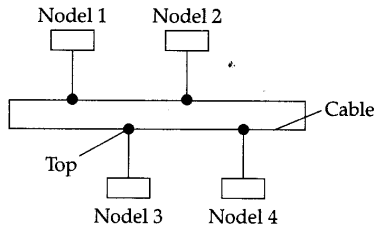Question 14:
Give one suitable example of each URL and Domain Name.
URL: http://waltons.in
Domain Name: @gmail.com

Question 15:
Write one advantage of star topology network? Also, illustrate how five (5) computers can be connected to each other using star topology of network.
Easy to replace, install or remove hosts or other devices.Question 16:
What is the function of Modem?
The function of modem is modulation and demo-dulation, means turn data into a format that can be transmitted via an audio link such as a phone line.

Question 17:
Explain the purpose of a router.
A router established connection between two networks and it can handle network with different protocols. Using a routing table, routers make sure that the data packets are travelling through the best possible paths to reach their destination.

Question 18:
Name any two components required for net-working.

1. Repeater
2. Routers

Question 19:
What are repeaters?
A repeater is an electronic device that receives a signal and retransmits it at a higher level and/ or higher power, or onto the other side of an obstruction, so that the signal can cover longer distances.

Question 20:
Mention one advantage of star topology over bus topology in networking?
Simple to add more computers to network.

Question 21:
Differentiate between packet swiching and message switching technique in network commu-nication.
In packet switched network, data are transmitted in discrete units of potentially variable length blocks called packets, while in message switching mechanism, a node receives a message stores it until the appropriate route is free, then sends it along.

Question 22:
Differentiate between Bus and Star topology of network.Question 23:
What is VoIP?
Voice over Internet Protocol (VoIP) is one of a family of internet technologies, communication protocols, and transmission technologies for delivery of voice communications and multi-media sessions over internet protocol (IP) network, such as the internet.

Question 24:
In networking, what is WAN? How is it different from LAN?
WAN (Wide Area Network):
A communication network that uses such devices as telephone lines, satellite, dishes, or radiowaves to span a larger geographic area which cannot be covered by a LAN.

Question 25:
Define the term bandwidth. Give any one unit of bandwidth.
Bandwidth is referred as the volume of information per unit of time that a transmission medium (like an internet connection) can handle.
OR
The amount of data that can be transmitted in a fixed amount of time is known as bandwidth. For digital devices, the bandwidth is usually expressed in bits per second (bps) or bytes per second. For analog devices, the bandwidth is expressed in cycles per second, or Hertz (Hz).

Question 26:
What is the difference between LAN and MAN?
LAN: It is Local Area Network. The diameter is not more than a single building.
WAN: It is Metropolitan Area Network. LAN spans a few kms while MAN spans 5-50 km diameter and is larger than a LAN.

Question 27:
What is the importance of URL in networking ?
A Uniform Resource Locator (URL) is used to specify, where an identified resource is available in the network and the mechanism for retrieving it. A URL is also referred to as a web address. 1

Question 28:
What was the role of ARPANET in the computer network?
In 1969, ARPANET was set up by the American government for defence purpose. ARPANET stands for Advanced research Projects agency Network.

Question 29:
Which of the following is not a unit for data transfer rate ?

1. bps
2. abps
3. gbps
4. kbps

(ii) abps

Question 30:
Identify the type of topology from the following:

1. Each node is connected with the help of a single cable
2. Each node is connected with the help of independent cable with central switching.

1. Bus topology
2. Star topology

Question 31:
ABC International School is planning to connect all computers, each spread over a distance of 50 metres. Suggest an economic cable type having high speed data transfer to connect these computers.
Optical fibre cable.

Question 32:
Mahesh wants to transfer data within a city at very high speed. Write the wired transmission medium and type of network.
Wired transmission medium – Optical fibre cable Type of network – MAN.

Question 33:
Which device is used to connect all computers inside a lab?
Hub

Question 34:
Which device is used to connect all computers to the internet using telephone wire?
RJ – 45. It is an eight wired connectors used to connect computers on a LAN.

Question 35:
What is Wi-Fi Card?
Wi-Fi cards are small and portable cards that allow the computer to connect to the internet through a wireless network. The transmission is through the use of radio waves.

Question 1:
Write any two differences between twisted pair and co-axial pair cable.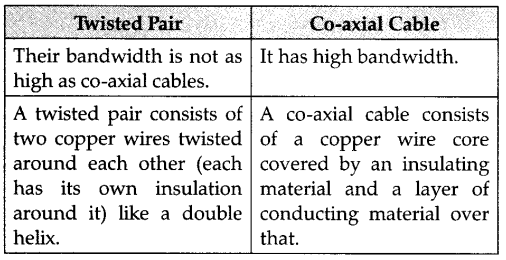Question 2:
Write one advantage of Bus Topology of network, also, illustrate how 4 computers can be connected with each other using star topology of network.
Bus topology: Cable length required for his topology is the least compared to other networks.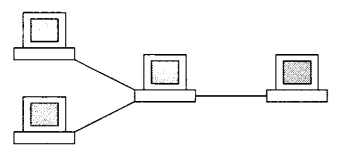Question 3:
Explain any two switching techniques used in networking.
Message Switching: It is similar to Post-office mailing system. A temporary link is established for one message transfer.
Packet Switching: It is a form of store and forward switching system which stores the message as small packets at the switch nodes and then transmits it to the destination.

Question 1:
(a) Differentiate between PAN and LAN types of networks.
(b) Which protocol helps us to transfer files to and from a remote computer ?
(c) Write two advantages of 3G over 2G mobile telecommunication technologies in terms of speed and services.
(d) Write two characteristics of Web 2.0
(e) Write the basic difference between worm and Trojan Horse.
(f) Categorize the following under client-side and server-side scripts category:

1. Jave Script
2. ASP
3. VB Script
4. JSP

(g)Intelligent Hub India is a knowledge community aimed to uplift the standard of skills and knowledge in the society. It is planning to setup its training centres in multiple towns and villages pan India with its head offices in the nearest cities. They have created a model of their network with a city, a town and 3 villages as given.
As a network consultant, you have to suggest the best network related solution for their issues/problems raised
in (i) to (iv) keeping in mind the distance between various locations and given parameters.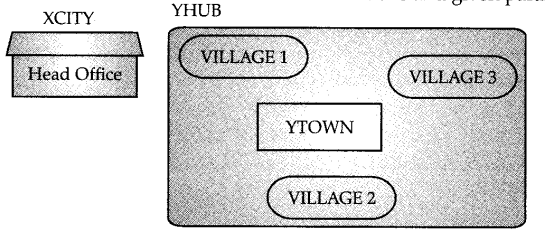Shortest distance between various locations: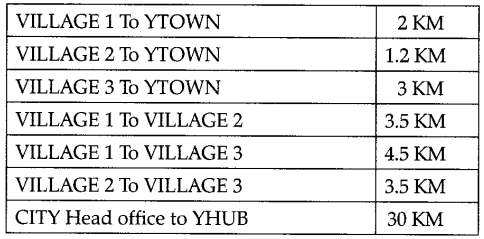Number of computers iinstalled at various locations are as follows:Note:
• In Villages, there are community centres, in which one room has been given as training center to this organiza¬tion to install computers.
• The organization has got financial support from the government and top IT companies.

2. Suggest the best wired medium and draw the cable layout (location to location) to efficiently connect vari¬ous locations within the YHUB.
3. Which hardware device will you suggest to connect all the computers within each location of YHUB?
4. Which server/protocol will be most helpful to conduct live interaction of Experts from Head office and people at YHUB locations?

1.(a)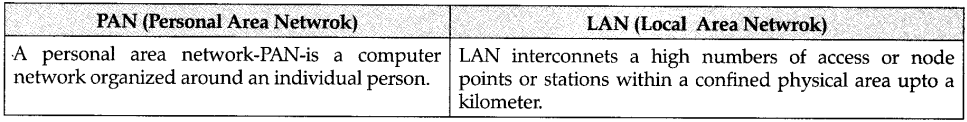OR
(b)
FTP or Telnet or TCP
FTP OR Telnet OR TCP
(c)
Speed

1. Faster web browsing.
2. Faster file transfer
Service
3. Better video clarity
4. Better security

OR
(Any other correct advantage can be considered)

(d) Makes web more interactive through online social media.
Supports easy online information exchange.
Interoperability on the internet.
Video sharing possible in the websites.
(e)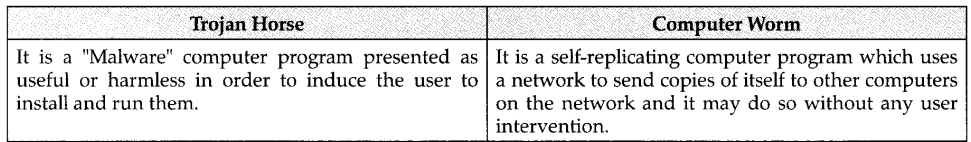(f)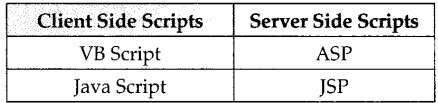(g)(i) YTOWN
Justification

1. Since it has the maximum number of computers.
2. It is closet to all other locatios.

(ii) Optical Fiber(iii) Switch or Hub
(iv) Video conferencing or VoIP or any other correct service/protocol.

Question 2:
Indian School, in Mumbai is starting up the network between its different wings. There are four Buildings named as SENIOR, JUNIOR, ADMIN and HOSTEL as shown below:The distance between various buildings is as follows:Number of Computers in Each Building :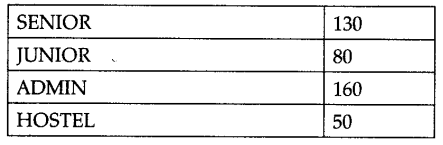1. Suggest the cable layout of connections between the buildings.
2. Suggest the most suitable place (i.e., building) to house the server of this school, provide a suitable reason.
3. Suggest the placement of the following devices with justification.
• Repeater
• Hub/Switch
4. The organisation also has inquiry office in another city about 50-60 km away in hilly region. Suggest the suitable transmission media to interconnect to school and inquiry office out of the following :
• Fiber optic cable
• Microwave

1.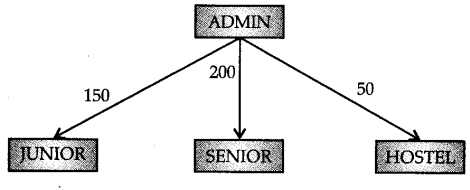2. Server can be placed in the ADMIN building as it has the maxium number of computer.
3. Repeater can be placed between ADMIN
and SENIOR building as the distance is more than 110 m.
4. Radiowaves can be used in hilly regions as they can travel through obstacles.

Question 3:
Vidya Senior Secondary Public School in Nainital is setting up the network between its different wings. There are 4 wings named as SENIOR(S), JUNIOR(J), ADMIN(A) and HOSTEL(H).
Distance between various wings are given below: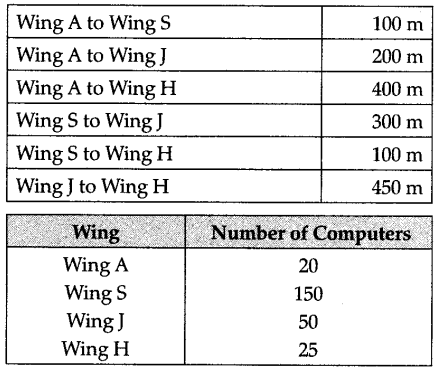1. Suggest a suitable Topology for networking the computers of all wings.
2. Name the most suitable wing where the Server should be installed. Justify your answer.
3. Suggest where all should Hub(s)/Switch(es) be placed in the network.
4. Which communication medium would you suggest to connect this school with its main branch in Delhi ?

1.2. Server should be in Wing S as it has the maxi-mum number of computers. 1
3. All Wings need hub/switch as it has more than
one computer.
4. Since the distance is more, wireless transmission would be better. Radiowaves are reliable and can travel through obstacles.

Question 5:

1. Which out ot three type of networks LAN. MAN and WAN, is to be used when an institute connects computers of two adjacent computer laboratories ?
2. What is the difference between HTTP and FTP?
3. What is the major difference between Message Switching and Packet Switching in networking?
4. What is the basic difference between DNS and URL?
5. Give two applications of web 2.0.
6. Categories the following under client side and Server-Side script category?
• JSP
• ASP
• VBScript
• Jave Script

(i) LAN (The ange is upto one KM)
(ii)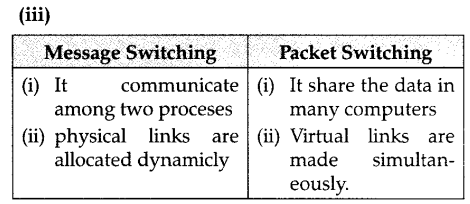(iv)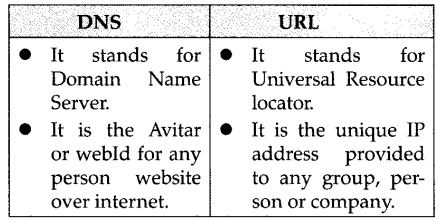(v) Application of web 2.0

1. web hosting
2. web browsing
3. web Indexing
4. web searching

(vi) Client side Script

1. JSP
2. Java Script
Server Side Scripts
3. ASP
4. VB Script

Question 6:
Trine Tech Corporation (TTC) is a professional consultancy company. The company is planning to set up their new offices in India with its hub at Hyderabad. As a network adviser, you have to understand their requirement and suggest them the best available solutions. Their queries are mentioned as (i) to (iv) below.
Physical Locations of the blocked of TTC1. What will be the most appropriate block, where TTC should plan to install their server?
2. Draw a block to cable layout to connect all the buildings in the most appropriate manner for efficient communication.
3. What will be the best possible connectivity out of the following, you will suggest to connect the new setup of offices in Bangalore with its London based office:
• Infrared
• Ethernet Cable
4. Which of the following device will be suggested by you to connect each computer in each of the buildings:
• Switch
• Modem
• Gateway

1. Finance block because it has maximum
number of computers.
2.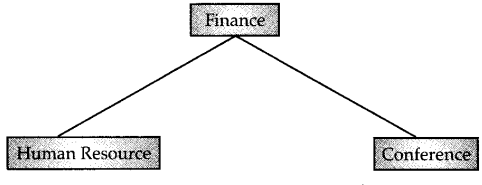4. Switch

Question 7:
G.R.K International Inc. is planning to connect its Bengaluru Office Setup with its Head Office in Delhi. The Bengaluru Office G.R.K. international Inc. is spread across and area of approx. 1 square kilometer, consisting of 3 blocks – Human Resources, Academics and Administration.
You as a network expert have to suggest answers to the four queries (i) to (iv) raised by them.
Notes : Keep the distance between blocks and number of computers in each block in mind, while providing them the solutions.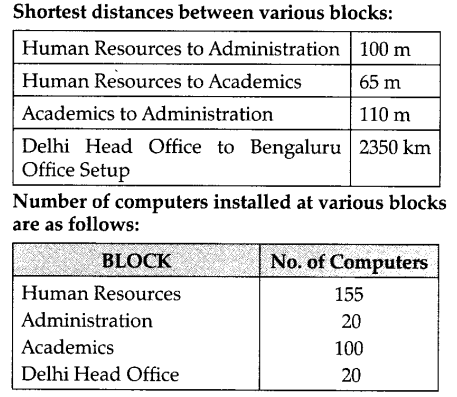1. Suggest the most suitable block in the Bengaluru Office Setup, to host the server.
Give a suitable reason with your suggestion.
2. Suggest the cable layout among the various blocks within the Bengaluru Office Setup for
connecting the Blocks.
3. Suggest a suitable networking device to be installed in each of the blocks essentially required for connecting computers inside the blocks with fast and efficient connectivity.
4. Suggest the most suitable media to provide secure, fast and reliable data connectivity between Delhi Head Office and the Bengaluru Office Setup.

1. Human Resources because it has maximum number of computers.
2.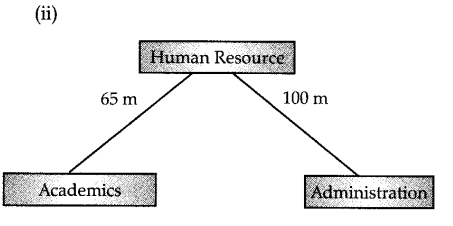3. Switch 1

Question 8:
Rovenza Communications International (RCI) is an online corporate training provider company for IT related courses. The company is setting up their new compus in Kolkata. You as a network expert have to study the physical locations of various blocks and the number of computers to be installed. In the planning phase, provide the best possible answers for the queries (i) to (iv) raised by them.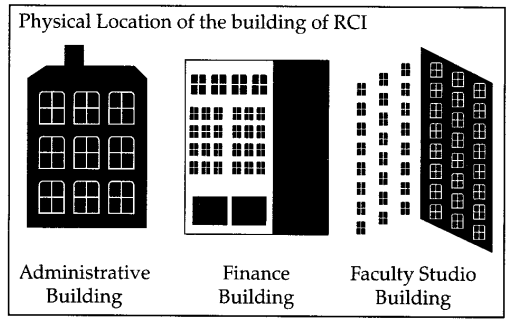Block to Block Distances(in Mtrs.)Expected computers to be installed in each block1. Suggest the most appropriate block, where RCI should plan to install the server.
2. Suggest the most appropriate block to block cable layout to connect all three blocks for efficient communication.
3. Which type of network out of the following is formed by connecting the computers of these three blocks?
• LAN
• MAN
• WAN
4. Which wireless channel out of the following should be opted by RCI to connect to students from all over the world?
• Infrared
• Microwave
• Satellite
5. Write two advantages of using open source software over proprietary software.
6. Which of the following crime(s) does not come under cybercrime?
• Copying some important data from a computer without taking permission from the owner of-the data.
• Stealing keyboard and mouse from a shop.
• Getting into unknown person’s social networking account and start messaging on his behalf.

1. Faculty Recording Block.
2. Star topology
3. LAN
4. Satellite connection
5. Advantages of open source over proprietary software:
• Open source software’s source code is available, can be modified copied & distributed while propritary software can’t be change.
• Open source is free while proprietary a paid.
6. (c) Stealing keyboard & mouse from a shop.4

Question 9:

1. Identify the type of topology on the basis of
the following:

• Since every node is directly connected to the server, a large amount of cable is needed which increases the installation cost of the network.
• It has a single common data path connecting all the nodes.

1. Star Topology
2. Bus Topology

(ii) Expand the following

1. VOIP
2. SMTP

1. Voice Over Internet Protocol
2. Simple Mail Transder Protocol

(iii) Who is a hacker?

A computer enthusiast, who uses his computer programming skill to intentionally access a computer without authorization is known as hacker. A hacker accesses the computer without the intention of destroying data or maliciously harming the computer.
(iv) The following is a 32 bit binary number usually represented as 4 decimal values, each representing 8 bits, in the range 0 to 255 (known as octets) separated by decimal points. 140.179.220.200
What is it? What is its importance?
It is an IP Address. It is used to identify the computers on a network.
(v) Daniel has to share the data among various computes of his two offices branches situated in the same city. Name the network (out of LAN, WAN, PAN and MAN) which is being formed in this process.
MAN
(vi)Rehaana Medicos Center has set up its new center in Dubai. It has four buildings as shown in the diagram given below: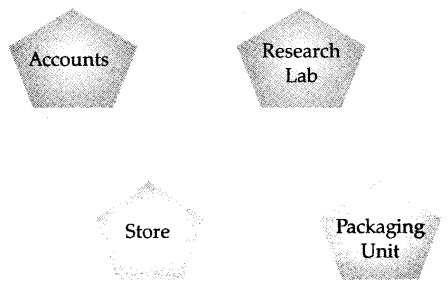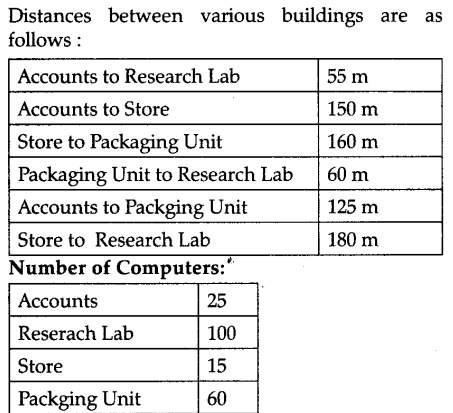As a network expert, provide the best possible answer for the following queries:

1. Suggest a cable layout of connections bet­ween the buildings.
2. Suggest the most suitable place (i.e. building) to house the server of this organization.
3. Suggest the placement of the following device with justification:
4. Repeater (b) Hub/Switch
5. Suggest a system (hardware/software) to prevent unauthorized access to or from the network.

1. Layout 1
2. The most suitable place / building to house the server of this organization would be building Research Lab, as this building contains the maximum number of computers.
3. Since the cabling distance between Accounts to Store is quite large, so a repeater would ideally be needed along their path to avoid loss of signals during the course of data flow in this route.
4. Firewall.Question 10:

1. What is the difference between domain name
2. Write two advantages of using an optical fibre cable over an Ethernet cable to connect two service stations, which are 190 m away from each other.
3. Expertfa Professsional Global (EPG) is an online, corporate training provider company for IT related courses. The company is setting up their new campus in Mumbai. You as a network expert have to study the physical locations of various buildings and the number of computers to be installed. In the planning phase, provide the best possible answer for theBuilding to Building distances (in Mtrs.)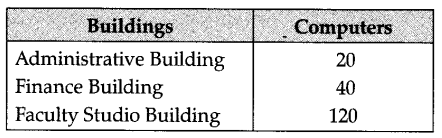1. Suggest the most appropriate building, where EPG should plan to install the server.
2. Suggest the most appropriate building to building cable layout to connect all three buildings for efficient communication.
3. Which type of network out of the following is formed by connecting the computers of these three buildings?
• LAN
• MAN
• WAN
4. Which wireless channel out of the following should be opted by EPG to connect to students of all over the world?
• Infrared
• Microwave
• Satellite

1. Domain Name is alphanumeric address
of a resource over network IP address is a Numeric Address of a resource in a Network.
Example:
Domain Name 1
www.Gabsclasses.com
102.112.0.153
• Faster Communication.
• Free from electrical & Noise interference.
3. (a) Faculty Studio Building
(b) Bus Topology
(c) LAN
(d) Satellite

Question 11:
To provide telemedicine faculty in a hilly state, a computer network is to be setup to connect hospitals in 6 small villages (VI, V2, …, V6) to the base hospital (H) in the state capital. This is shown in the following diagram.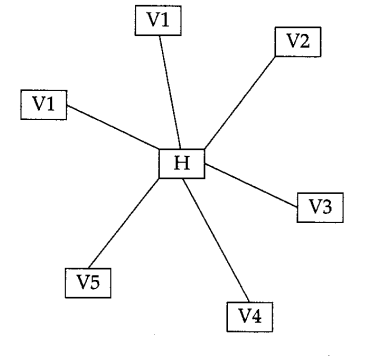No village is more than 20 km away from the state capital.
Imagine yourself as a computer consultant for this project and answer the following questions with justification:

2. What kind of network will be formed; LAN, MAN, or WAN ?
3. Many times doctors at village hospital will have to consult senior doctors at the base hospital. For this purpose, how should they contact them: using email, sms, telephone, or video conference ?

(b) Out of SMTP and POP3 which protocol is used to receive emails ?
(c) What are cookies in the context of computer networks?
(d) Rajeshwari is trying for on-line subscription to a magazine. For this she has filled in a form on the magazine’s web site. When the clicks submit button she gets a message that she has left e-mail field empty and she must fill it. For such checking which type of script is generally executed client side script or server-side script ?
(e) Mention any one difference between free-ware and free software.

(a)

2. MAN
3. e-mail

(b) POP3
(c) Cookies are files that store user information that is used to identify the user when he logs into the system.
(d) Server-side script
(e) Freeware is a software that has the user to get unlimited usage for. Free software may be free for a certain period only.

Question 12:
Workalot Consultants are setting up a secured network for their office campus at Gurgaon for their day-to-day office and web-based activities. They are planning to have connectivity between three buildings and the head office situated in Mumbai. Answer the questions (i) to (iv) after going through the building positions in the campus and other details, which are given below :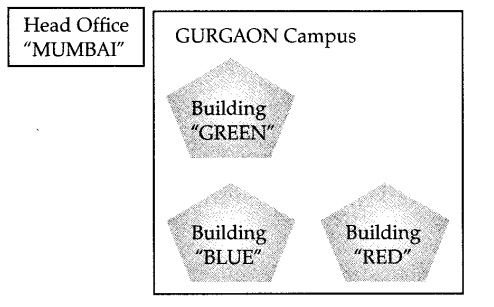1. Suggest the most suitable place (i.e., building) to house the server of this organization. Also give a reason to justify your suggested location.
2. Suggest a cable layout of connections between the buildings inside the campus.
3. Suggest the placement of the following devices with justification:
• Repeater.
• Switch.
4. The organization is planning to provide a high speed link with its head office situated in Mumbai using a wired connection. Which of the following cables will be most suitable for this job ?
• Optical Fiber
• Co-axial Cable
• Ethernet Cable

1. The most suitable place to install server is building “RED” because this building have maximum computer which reduce communication delay.2. (a) Since the cabling distance between buildings GREEN, BLUE and RED are quite large, so a repeater each, would ideally be need along their path to avoid loss of signals during the course of data flow in there routes.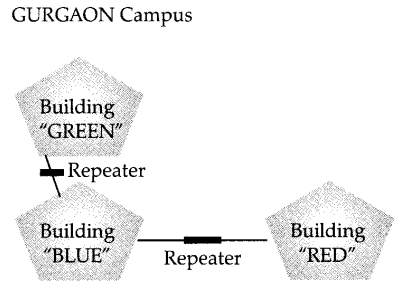(b) In the layout a switch each, would be needed in all the buildings, to interconnect the group of cables from the different computers in each building.(iv) Optical fiber

Question 13:
Granuda Consultants are setting up a secured network for their office campus at Faridabad for their day to day office and web based activities. They are planning to have connectivity between 3 building and the head office situated in Kolkata. Answer the questions (i) to (iv) after going through the building positions in the campus and other details, which are given below:1. Suggest the most suitable place (i.e., block) to house the server of this organization. Also give a reason to justify your suggested location.
2. Suggest a cable layout of connections between the buildings inside the campus.
3. Suggest the placement of the following devices with justification:
• Repeater
• Switch
4. The organization is planning to provide a high speed link with its head office situated in the  KOLKATA using a wired connection. Which of the following cable will be most suitable for this job?
• Optical Fibre
• Co-axial Cable
• Ethernet Cable

1. The most suitable place to install server is building “JAMUNA” because this building have maximum computer which reduce the communication delay.
2. Cable layout. (Bus topology).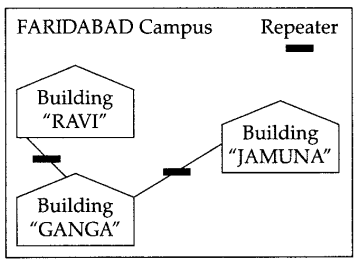3. (a) Since the cabling distance between buildings GANGA and JAMUNA are quite large, so a repeater each, would ideally be needed along their path to avoid loss of signals during the course of data flow in these routes.(b) In the layout a switch each would be needed in all the building, to interco¬nnect the group of cables from the different computers in each building.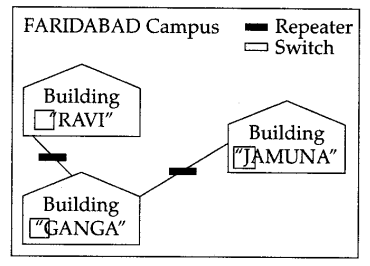4. Optical fiber

Question 14:
India Skills Hub is a skill development community which has an aim to promote the standard of skills in the society. It is planning to set up its training centres in multiple towns and villages Pan India with its head offices in the nearest cities. They have created a model of their network with a city ABC Nagar, a town (UVW town) and 3 villages.
As a network consultant, you have to suggest the best network related solutions for their issues/ problems raised in (i) to (iv), keeping in mind the distances between various locations and other given parameters.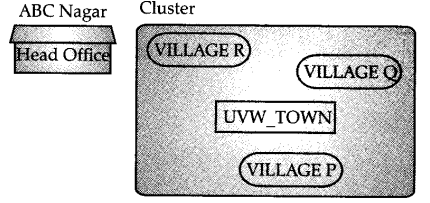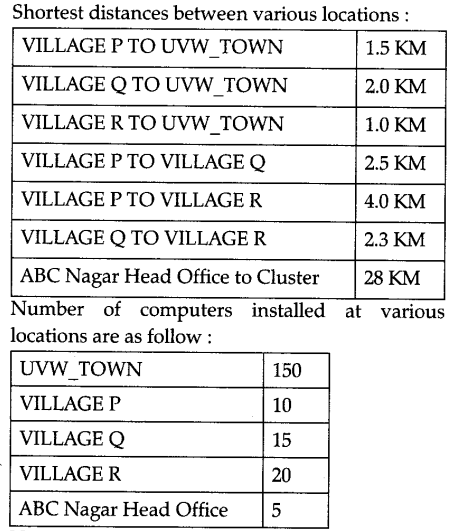Note:
In Villagers, there are community centers, in which one room has been given as training entrer to this organization to install computers.
The organization has got financial support from the government and top Multinational Organi­zations.

2. Suggest the best wired medium and draw the cable layout (location to location) to efficiently connect various locations within the Cluster.
3. Which hardware device will you suggest to connect all the computers within each location of
4. Which service/protocol will be most helpful to conduct live interactions of Expersts from Head Office and peole at all locations of Cluster?

1. Best location for the server is UVW-TOWN, because it is approximately equidistant from the village P, Q and R.
2. For connectivity between UVW-TOWN to head office is optic Fiber and to connect the villages, P, Q and R with server at UVW- TOWN is co-axial cable.
3. The villages R Q and R can be connected with server at UVW-TOWN by a Hub and the head office is connected by a Bus topology.
4. Between head office and UVWTOWN
we recommend for Bus topology, so HTTP protocol and other terminal can be connected by UDP or FTP protocols.

Question 15:
Mudra publishing is a group of companies engaged in publishing IT related books located in the hilly area of Shimla. The companies are located in four different, blocks whose layout is shown in the following figure. Answer the questions (i) to (iv) with the relevant justifi-cations.
Mudra publishing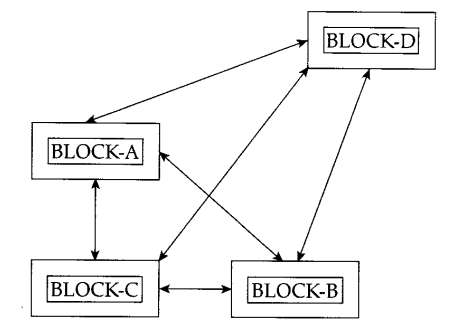Distance between various Blocks :

1. Block A to Block C is 50 m
2. Block A to Block D is 100 m
3. Block B to Block C is 40 m
4. Block B to Block D is 70 m
5. Block C to Block D is 125 m
Number of Computers
6. Block A is 25
7. Block B is 50
8. Block C is 20
9. Block D is 120

1. Suggest a suitable network topology between the blocks.
2. Which is the most suitable block to house the server of this organization?
3. Suggest the placement of the following devices with justification
• Repeater
• Switch
4. The organization is planning to link the whole blocks to its marketing Office in Delhi. Since cable connection is not possible from Shimla, suggest a way to connect it with high speed.

1. Suitable topology is bus topology.
2. The most suitable block for hosting server is BLOCK-D because this block has maximum number of computers.
Mudra Publishing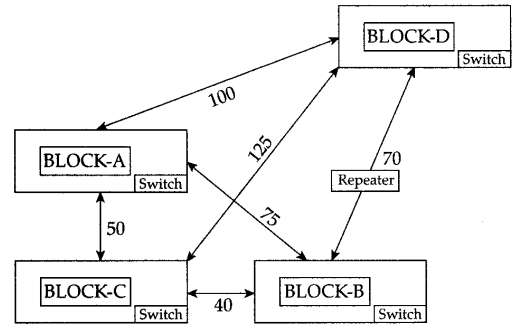3. Switch is a device used to segment network into different sub-networks so switch will exist in all the blocks. Since distance between BLOCK-D and BLOCK-C is large so repeater will be install between BLOCK-D and BLOCK-C.
4. The most economic way to connect it with a reasonable high speed would be the use radiowave transmission, as they are easy to install, can travel long distance and penetrate buildings easily, so they are used for communication, both indoors and outdoors. Radiowaves also have the advantage of being omni-directional. They can travel in all the directions from the source, so that the transmitter and receiver do not have to be carefully aligned physically.

Topic 2

Question 1:
Define firewall.
A system designed to prevent unauthorized access to or from a private network is called firewall, it can be implemented in both hardware and software or combination of both.

Question 2:
Write any two examples of Server side Scripts.

1. ASP
2. PHP

Question 3:
What is the difference between E-mail & chat?

1. Chat occurs in near real time, while E-mail doesn’t.
2. Chat is a 2-way communication which require the permission of both parties, while E-mail is a 1-way communication.

Question 4:
Write names of any two popular open source software, which are used as operating systems.

1. Kernels
2. Unix
3. Linux

Question 5:
What is the difference between video conferencing and chat.
In conference, we can include more than one person and it allows text video and audio while chat is one-to-one communication.

Question 6:
Expand the following abbreviations :

1. HTTP
2. VOIP

1. HTTP-Hyper Text Transfer Protocol.
2. VOIP-Voice Over Internet Protocol.

Question 7:
What is the difference between HTTP and FTP?
Hyper Text Transfer Protocol deals with Transfer of Text and Multimedia content over internet while FTP (File Transfer Protocol) deals with transfer of files over the internet.

Question 8:
What out of the following, will you use to have an audio-visual chat with an expert sitting in a far-away place to fix-up a technical issue ?

1. VoIP
2. email
3. FTP

(i) VoIP 1

Question 9:
Name one server side scripting language and one client side scripting language.
Client Side :

1. JAVASCRIPT
2. VBSCRIPT
Server Side
3. ASP
4. JSP

Question 10:
Which out of the following comes under cyber crime ?

1. Operating someone’s internet banking account, without his knowledge.
2. Stealing a keyboard from someone’s computer.
3. Working on someone’s Computer with his/ her permission.

(i) Operating someone’s internet banking account, without his knowledge.

Question 11:
Name two proprietary software along with their application.

1. MS-Office – All office applications MS-Word, MS-Excel, MS-PowerPoint.
2. Visual Studio – Visual Basic, Visual C+ +
software for application development.

Question 12:
Out of the following, identify client side script (s) and server side script (s).

1. ASP
2. Javascript
3. VBScript
4. JSP

Server side scripting

1. ASP
2. JSP
Client side scripting
3. Javascript
4. VBscript Vi

Question 13:
Compare open source software and proprietary software.
One need an authorized license paid in order to use this proprietary software while open source
software can be used by anybody and are usually free. One can use this software for their personal use. After giving any notation or code in this type one cannot change the code or modify it.

Question 14:
Differentiate between XML and HTML.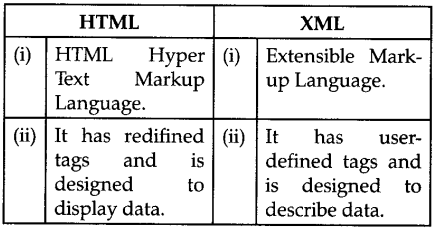Question 15:
What is WEB 2.0 ?
Web2.0 refers to the new generation of web based services and communities characterised by participation, collaboration and sharing of information among users online.

Question 16:
Which of the following is/are not a client side script:

1. VB Script
2. Java Script
3. ASP
4. PHP ?

(iii) ASP and
(iv) PHP are not client side scripts.

Question 17:
If someone has hacked your website, to whom you lodge the complaint ?
The complaint has to be lodged with the police under IT Act.

Question 18:
What do you mean by IP address? How is it useful in computer security ?
An Internet Protocol (IP) address is a numerical identification and logical address that is assigned to devices connected in a computer network.
An IP address is used to uniquely identify devices on the internet and so one can quickly know the location of the system in the network. 1

Question 19:
What do you mean by spam mails ? How can you protect your mailbox from spams ?
Spam mails, also known as junk e-mail, is a subset of spam that involves nearly identical messages sent to numerous recipients by e-mail.
We can protect our mailbox from spams by creating appropriate filters.

Question 20:
When do you prefer XML over HTML and why ?
The first benefit of XML is that because you are writing your own markup language, you are not restricted to a limited set of tags defined by proprietary vendors.
Rather than waiting for standards bodies to adopt tag set enhancements (a process which can take quite some time), or for browser companies to adopt each other’s standards with XML, you can create your own set of tags at your own place.

Question 21:
How does firewall protect our network ?
A firewall is a part of a computer system or network, that is designed to block unauthorized access while permitting authorized communi-cations. It is a device or set of devices configured to permit, deny, encrypt, decrypt, or proxy all (in and out) computer traffic between different security domains based upon a set of rules and, other criteria. 1

Question 22:
Compare freeware and shareware.
Freeware, the name derived from the words “free” and “software”. It is a computer software that is available for use at no cost or for an optional fee. Freeware is generally proprietary software available at zero price, and is not free software. The author usually restricts one or more rights to copy, distribute, and make derivative works of the software.
Shareware is usually offered as a trial version with certain features only available after the license is purchased, or as a full version, but for a trial period. Once the trial period has passed, the program may stop running until a license is purchased. Shareware is often offered without support, updates, or help menus, which only become available with the purchase of a license. The words “free trial” or “trial version” are indicative of shareware.

Question 23:
How trojan horses are different from worms? Mention any one difference.
A trojan horse is a term used to describe malware that appears to the user, to perform a desirable function but, in fact, facilitates unauthorized access, to the user’s computer system.
A computer worm is a self-replicating computer program. It uses a network to send copies of itself to the other nodes (computers on the network) and it may do so without any user intervention.

Question 24:
Give the full form of :

1. FOSS
2. HTTP

1. FOSS : Free Open Source Software.
2. HTTP : Hyper Text Transfer Protocol.

Question 25:
What is the difference between trojan horse and virus in terms of computers ?
A trojan, sometimes referred to as a trojan horse, is non-self-replicating malware that appears to perform a desirable function for the user but instead facilitates unauthorized access to the user’s computer system. The term is derived from the trojan horse story in greek mythology.
A computer virus is a computer program that can copy itself and infact a computer. A true virus can only spread from one computer to another.
The chief difference between a trojan horse and a virus is that a trojan horse does not replicate itself. A virus does. 1

Question 26:
Which term we use for a software/hardware device, which is used to block, unauthorized access while permitting authorized communi¬cations. This term is also used for a device or set of devices configured to permit, deny, encrypt, decrypt, or proxy all (in and out) computer traffic between different security domains based upon a set of rules and other criteria.
Firewall.

Question 27:
Write the full forms of the following:

1. GNU
2. XML

1. GNU stands for “GNU’s Not Unix”
2. XML stands for “Extensible Markup Language”.

Question 28:
Expand the following terminologies:

1. GSM
2. WLL (IMP)

1. GSM: Global System for Mobile Communication
2. WLL: Wireless Local Loop.

Question 29:
Name some cyber offences under the IT Act.

1. Tampering with computer source documents
2. Hacking.
3. Publishing of information which is obscene
in electronic form.

Question 30:
What are the three ways of protecting intellectual property ?

1. Patents

Question 31:
When a user browses a website, the web server sends a text file to the web browser. What is the name of this?

Question 1:
Define the following:

1. Firewall
2. VoIE

1. Firewall: A system designed to prevent
unauthorized access to or from a private network is called firewall, it can be implemented in both hardware and software or combination of both.
2. VoIP: Voice-over-Internet Protocol(VoIP) is a methodology and group of technologies for the delivery of voice communications and multimedia sessions over Internet Protocol (IP) networks, such as the Internet.

Question 2:
Give the full form of the following terms.

1. XML
2. FLOSS
3. HTTP
4. FTP

1. XML: Extensible Markup Language.
2. FLOSS: Free-Libre Open Source Software.
3. HTTP: Hyper Text Transfer Protocol.
4. FTP: File Transfer Protocol.

Question 3:
Differentiate between WORM and VIRUS in Computer terminology.
VIRUS directly effects the system by corrupting the useful data. A computer virus attaches itself to a program or file enabling it to spread from one computer to another.
A WORM is similar to a virus by design is considered to be subclass of a virus. Worm spread from computer to computer, but unlike a virus, it has the capability to travel without any human action. 2

Question 4:
Expand the following

1. GSM
2. GPRS.

1. GSM: Global System for Mobile Communi-cation.
2. GPRS: General Packet Radio Service.

Question 5:
Expand the following abbreviations :

1. HTTP
2. VOIP

1. HTTP: Hyper Text Transfer Protocol.
2. VOIP: Voice Over Internet Protocol.

Question 6:
Give the full form of :

1. FOSS
2. HTTP

1. FOSS: Free Open Source Software.
2. HTTP: Hyper Text Transfer Protocol.

Question 7:
Write the full forms of the following :

1. GNU
2. XML

1. GNU: GNU’s Not Unix
2. XML: Xtensible Markup Language.

Question 8:
Expand the following terminologies :

1. GSM
2. WLL

1. GSM: Global System for Mobile Communi-cation
2. WLL: Wireless Local Loop.

Question 9:
Give the full forms of the following terms :

1. CDMA
2. TDMA
3. FDMA

1. CDMA: Code Division Multiple Access
2. TDMA: Time Division Multiple Access
3. FDMA: Frequency Division Multiple Access

Question 10:
Expand the following abbreviations :

1. FTP
2. TCP
3. SMTP
4. VOIP

1. FTP: File Transfer Protocol
2. TCP: Transmission Control Protocol.
3. SMTP: Simple Mail Transfer Protocol.
4. VOIP: Voice Over Internet Protocol

## Important Questions for Class 12 Computer Term 2 with Answers: Database Management

Database concepts

Question 1:
Observe the following table MEMBER carefully and write the name of the RDBMS operation out of (i) SELECTION (ii) PROJECTION (iii) UNION (iv) CARTESIAN PRODUCT, which has been used to produce the output as shown in RESULT. Also, find the Degree and Cardinality of the RESULT: All India 2017
RDBMS Operation → SELECTION
Degree of table RESULT = 3
Cardinality of table RESULT = 1

Question 2:
Observe the following PARTICIPANTS and EVENTS tables carefully and write the name of the RDBMS operation which will be used to produce the output as shown in RESULT? Also, find the Degree and Cardinality of the RESULT. All India 2016
RDBMS Operation → Cartesian Product
Degree → 4, Cardinality → 6

Question 3:
Observe the following STUDENTS and EVENTS tables carefully and write the name of the RDBMS operation which will be used to produce the output as shown in LIST. Also, find the Degree and Cardinality of the LIST. Delhi 2016
RDBMS Operation → Cartesian Product
Degree → 4, Cardinalitv → 6

Question 4:
Observe the following table carefully and write the names of the most appropriate columns, which can be considered as
(i) Candidate keys and (ii) Primary key. Delhi 2015
(i) Candidate Keys → Id, Product
(ii) Primary Key → Id

Question 5:
Observe the following table carefully and write the names of the most appropriate columns, which can be considered as (i) Candidate keys and (ii) Primary key: All India 2015
(i) Candidate Keys → Code, Item
(ii) Primary Key → Code

Question 6:
Observe the following table carefully and find the degree and cardinality of the table : All India 2018 (C)
Degree → 3, Cardinality → 5

Question 7:
Observe the following table and answer the parts (i) and (ii): All India 2014 (C)
(i) In the above table, can we have Qty as primary key? [Answer as yes/no]. Justify your answer.
(ii) What is the cardinality and degree of the above table?
(i) No, Qty cannot have as primary key because it is not uniquely identify in a table.
(ii) Cardinality → 5, Degree → 4

Question 8:
Explain the concept of Cartesian Product between two tables, with the help of appropriate example. All India 2014

Or

Illustrate cartesian product operation between the two tables/relations using a suitable example. Delhi 2012 (C)
Cartesian Product The cartesian product means the cross join of two relations and the resultant relation will contain all the possible combinations of the tuples from the two tables, i.e. the cardinality of the resulting relation will be equal to the product of cardinalities of the two relations. It is denoted by ‘x’ symbol.
Question 9:
Explain the concept of candidate keys with the help of an appropriate example. All India 2013

Or

What do you understand by candidate key in a table? Give a suitable example of candidate key from a table containing some meaningful data. Delhi 2010.2009
A candidate key is a set of one or more attributes that uniquely identify in a table. There can be multiple candidate keys in one table. Each candidate key can work as a primary key.

Question 10:
What is the difference between degree and cardinality of a table? What is the degree and cardinality of the following table?
Delhi 2013
Degree The number of attributes or columns in a table is called the degree of the table. The degree of the given table is : 3.
Cardinality The number of rows or records in a table is called ,the cardinality of the table. The cardinality of the given table is : 2.

Question 11:
Differentiate between the primary key and alternate key of a table with the help of an example. Delhi 2013C

Or

Give a suitable example of a table with sample data and illustrate primary and alternate keys in it. All India 2012.2011; Delhi 2011 (c)
Primary Key It’s a column or set of columns that helps to identify records uniquely. One table can have only one primary key.
Alternate Key/Secondary Key It’s a column or set of columns that can act as a primary key but not selected as a primary key.
e.g. In table Student, RollNo and AdmNo of all students are different.
Both of them can be selected as primary key. Suppose we have selected RollNo as a primary key then AdmNo is called as alternate key.

Question 12:
Give suitable example of a table with sample data and illustrate primary and candidate keys in it. Delhi 2012
The table student is as follows:
Here, AdmNo and RollNo both can identify records uniquely. So, both are candidate keys, and from them we can also make AdmNo as a primary key.

Question 13:
What do you mean by union and cartesian product operation in relational algebra? Delhi 2011
Union (binary operator) It operates on two relations and is indicated by ‘∪’.
Cartesian Product (binary operator) It operates on two relations and is denoted by ‘×’. e.g. cartesian product of two relations R1 and R2 is represented by R = R1 x R2. The degree of R is equal to sum of degrees of R1 and R2. The cardinality of R is product of cardinality of R1 and cardinality of R2.
e.g. R = R1 ∪ R2 represents union operation between two relations R1 and R2. The degree of R is equal to degree of R1. The cardinality of R is sum of cardinality of R1 and cardinality of R2.

Question 14:
What do you understand by selection and projection operation in relational algebra? All India 2011

Question 15:
What do you understand by primary key? Give a suitable example of primary key from a table containing some meaningful data. All India 2010
A primary key is a set of one or more attributes that can uniquely identify tuples within the relation.
e-g.
The attribute ItemNo is a primary key as it contains unique value for each tuple in a relation.

Question 16:
What is the purpose of a key in a table? Give an example of a key in a table. All India 2009
A key is used to identify a tuple uniquely within the relation. The value of key is unique. No rows in the relation can have same value, e.g. in an Employee relation EmpCode is a key, using EmpCode one can obtain the information of a particular employee.

## Important Questions for Class 12 Computer Term 2 with Answers: Structured Query Language

Question 1:
Explain the concept UNION between two tables, with the help of appropriate example. Delhi 2014
The UNION operator is used to combine the result-set of two or more tables, without returning any duplicate rows,
Question 2:
Write SQL queries for (i) to (iv) and find outputs for SQL queries (v) to (viii), which are based on the tables.All India 2017
(i) To display all details from the table MEMBER in descending order of ISSUEDATE.
(ii) To display the DCODE and DTITLE of all Folk Type DVDs from the table DVD.
(iii) To display the DTYPE and number of DVDs in each DTYPE from the table DVD.
(iv) To display all NAME and ISSUEDATE of those members from the table MEMBER who have DVDs issued
(i.e., ISSUEDATE) in the year 2017.

(v) SELECT MIN (ISSUEDATE) FROM MEMBER;
(vi) SELECT DISTINCT DTYPE FROM DVD;
(vii) SELECT D.DCODE, NAME, DTITLE '
FROM DVD D, MEMBER M WHERE D.DC0DE=M.DCODE;
(viii) SELECT DTITLE FROM DVD
WHERE DTYPE NOT IN ("Folk”, "Classical”);

(i) SELECT * FROM MEMBER ORDER BY ISSUEDATE DESC;
(ii) SELECT DCODE, DTITLE FROM DVD WHERE DTYPE = "Folk";
(iii) SELECT DTYPE, COUNT (*) FROM DVD GROUP BY DTYPE;
(iv) SELECT NAME, ISSUEDATE FROM MEMBER WHERE ISSUEDATE LIKE ‘2017%’;

Question 3:
Write SQL queries for (i) to (iv) and find outputs for SQL queries (v) to (viii),
which are based on the tables. All India 2016

NOTE

• KM is Kilometres travelled
• NOP is number of passengers travelled in vehicle.

(i) To display CNO, CNAME, TRAVELDATE from the table TRAVEL in descending order of CNO.
(ii) To display the CNAME of all the customers from the table TRAVEL who are traveling by vehicle with code V01 or V02.

(iii) To display the CNO and CNAME of those customers from the table TRAVEL who travelled between ‘2015-12-31’ and ‘2015-05-01’.
(iv) To display all the details from table TRAVEL for the customers, who have travel distance more than 120 KM in ascending order of NOP.

(v) SELECT COUNT(*), VCODE FROM TRAVEL
GROUP BY VCODE HAVING C0UNT(*)>1;
(vi) SELECT DISTINCT VCODE FROM TRAVEL;
(vii) SELECT VCODE,CNAME,VEHICLETYPE
FROM TRAVEL A, VEHICLE B
WHERE A.VC0DE=B.VCODE AND KM<90;
(viii) SELECT CNAME, KM*PERKM
FROM TRAVEL A, VEHICLE B
WHERE A.VC0DE=B.VCODE AND A.VC0DE='V05';

(i) SELECT CNO, CNAME, TRAVELDATE FROM TRAVEL ORDER BY CNO DESC;
(ii) SELECT CNAME FROM TRAVEL WHERE VCODE = "VO1" OR VC0DE="VO2";
(iii) SELECT CNO, CNAME FROM TRAVEL WHERE TRAVELDATE BETWEEN '2015-12-31' AND ‘2015-05-01 ‘;
(iv) SELECT * FROM TRAVEL WHERE KM>120  ORDER BY NOP

Question 4:
Write SQL queries for (i) to (iv) and find outputs for SQL queries (v) to (viii), which are based on the tables. Delhi 2016
NOTE
• PERKM is Freight Charges per kilometre • VTYPE is Vehicle Type

NOTE
• NO is Traveller Number
• KM is Kilometre travelled
• NOP is number of travellers travelled in vehicle
• TDATE is Travel Date

(i) To display NO, NAME, TDATE from the table TRAVEL in descending order of NO.
(ii) To display the NAME of all the travellers from the table TRAVEL who are travelling by vehicle with code 101 or 102. ‘
(iii) To display the NO and NAME of those travellers from the table TRAVEL who travelled between ’2015-12-31’ and ‘2015-04-01’.

(iv) To display all the details from table TRAVEL for the travellers, who have travelled distance more than 100 KM in ascending order of NOP.

(v) SELECT COUNT(*), CODE FROM TRAVEL
GROUP BY CODE HAVING C0UNT(*) >1;
(vi) SELECT DISTINCT CODE FROM TRAVEL;
(vii) SELECT CODE,NAME,VTYPE
FROM TRAVEL A, VEHICLE B
WHERE A.C0DE=B.C0DE AND KM<90;
(viii) SELECT NAME,KM*PERKM
FROM TRAVEL A, VEHICLE B
WHERE A.C0DE=B.C0DE AND A.C0DE='105' ;

(i) SELECT NO, NAME, TDATE FROM TRAVEL ORDER BY NO DESC;
(ii) SELECT NAME FROM TRAVEL WHERE CODE = 101 OR CODE = 102;
(iii) SELECT NO. NAME FROM TRAVEL WHERE TDATE BETWEEN '2015-12-31' AND '2015-04-01' ;
(iv) SELECT * FROM TRAVEL WHERE KM > 100 ORDER BY NOP;

Question 5:
Consider the following DEPT and WORKER tables.
Write SQL queries for (i) to (iv) and find outputs for SQL queries (v) to (viii): Delhi 2015

NOTE
DOJ refers to Date of Joining and DOB refers to Date of Birth of workers.
(i) To display WNO, NAME,, GENDER from the table WORKER in descending order of WNO.
(ii) To display the NAME of all the FEMALE workers from the table WORKER.
(iii) To display the WNO and NAME of those workers from the table WORKER, who are born between ‘1987-01-01’ and ‘1991-12-01’.
(iv) To count and display MALE workers who have joined after ‘1986-01-01’.

(v) SELECT COUNT(*), DCODE FROM WORKER
GROUP BY DCODE HAVING C0UNT(*)>1;
(vi) SELECT DISTINCT DEPARTMENT FROM DEPT;
(vii) SELECT NAME, DEPARTMENT, CITY FROM WORKER W, DEPT D
WHERE W.DC0DE=D.DCODE AND WNO<1003;
(viii) SELECT MAX (DOJ), MIN(DOB) FROM WORKER;

(i) SELECT WNO, NAME, GENDER FROM WORKER ORDER BY WNO DESC;
(ii) SELECT NAME FROM WORKER WHERE GENDER = "FEMALE";
(iii) SELECT WNO, NAME FROM WORKER WHERE DOB BETWEEN '1987-01-01' AND '1991-12-01';
(iv) SELECT COUNT(*) FROM WORKER WHERE GENDER = "MALE" AND DOJ > '1986-01-01';

Question 6: Consider the following DEPT and EMPLOYEE tables.
Write SQL queries for (i) to (iv) and find outputs for SQL queries (v) to (viii). All India 2015

NOTE DOJ refers to Date of Joining and DOB refers to Date of Birth of employees.
(i) To display ENO, NAME, GENDER from the table EMPLOYEE in ascending order of ENO.
(ii) To display the NAME of all the MALE employees from the table EMPLOYEE.
(iii) To display the ENO and NAME of those employees from the table EMPLOYEE who are born between ‘1987-01-01’ and ‘1991-12-01’.
(iv) To count and display FEMALE employees who have joined after ‘1986-01-01’.

(v) SELECT COUNT (*), DC0DE FROM EMPLOYEE
GROUP BY DCODE HAVING C0UNT(*)>1;
(vi) SELECT DISTINCT DEPARTMENT FROM DEPT;
(vii) SELECT NAME, DEPARTMENT FROM EMPLOYEE E.DEPT D
WHERE E.DCODE = D.DCODE AND ENO<1003;
(viii) SELECT MAX(DOJ),MIN(DOB)FROM EMPLOYEE;

(i) SELECT ENO, NAME, GENDER FROM EMPLOYEE ORDER BY ENO;
(ii) SELECT NAME FROM EMPLOYEE WHERE GENDER = 'MALE';
(iii) SELECT ENO, NAME FROM EMPLOYEE WHERE DOB BETWEEN '1987-01-01' AND '1991-12-01';
(iv) SELECT COUNT!*) FROM EMPLOYEE WHERE GENDER = 'FEMALE' AND DOJ >'1986-01-01' ;

Question 7:
Consider the following tables SCHOOL and ADMIN  and answer (a) and (b) parts of this question : All India 2014

(a) Write SQL statements for the following:
(i) To display TEACHERNAME, PERIODS of all teachers whose periods are more than 25.
(ii) To display all the information from the table SCHOOL in descending order of experience.
(iii) To display DESIGNATION without duplicate entries from the table ADMIN.
(iv) To display TEACHERNAME, CODE and corresponding DESIGNATION from tables SCHOOL and ADMIN of Male teachers.
(b) Give the output of the following SQL queries :

(i) SELECT DESIGNATION, COUNT (*) FROM ADMIN GROUP BY DESIGNATION HAVING COUNT (*)<2;
(ii) SELECT MAX (EXPERIENCE) FROM SCHOOL;
(iii) SELECT TEACHERNAME FROM SCHOOL WHERE EXPERIENCE > 12 ORDER BY TEACHERNAME;
(iv) SELECT COUNT (*), GENDER FROM ADMIN GROUP BY GENDER;

(a) (i) SELECT TEACHERNAME, PERIODS
FROM SCHOOL WHERE PERI0DS>25;
(ii) SELECT *FROM SCHOOL ORDER BY EXPERIENCE DESC;
(iii) SELECT DISTINCT DESIGNATION FROM ADMIN;
(iv) SELECT TEACHERNAME, CODE, DESIGNATION FROM SCHOOL S,
ADMIN A WHERE S.CODE = A.CODE AND  GENDER = "MALE";
Question 8:
Answer the questions (a) and (b) on the basis of the following tables STORE and ITEM. Delhi 2014

(a) Write the SQL queries
(i) to (iv): (i) To display IName and Price of all the Items in ascending order of their Price.
(ii) To display SNo and SName of all Store located in CP.
(iii) To display Minimum and Maximum Price of each IName from the table ITEM.
(iv) To display IName, Price of all items and their respective SName where they are available.
(b) Write the output of the following SQL commands (i) to (iv):

(i) SELECT DISTINCT IName FROM ITEM
WHERE Price >=5000;
(ii) SELECT Area, COUNT(*)
FROM STORE GROUP BY Area;
(iii) SELECT COUNT(DISTINCT Area) FROM STORE;
(iv) SELECT IName, Price * 0.05 DISCOUNT FROM ITEM
WHERE SNo IN (S02, S03);

(a)(i) SELECT IName, Price FROM ITEM ORDER BY Price;
(ii) SELECT SNo, SName FROM STORE WHERE Area = 'CP’;
(iii) SELECT IName, MIN(Price)"Minimum Price", MAX(Price)"Maximum Price" FROM ITEM GROUP BY IName;
(iv) SELECT IName, Price, SName FROM ITEM I, STORE S WHERE I.SNo = S.SNo;

Question 9:
Answer the questions (a) and (b) on the basis of the following tables
SHOPPE and ACCESSORIESAll India 2014

(a) Write the SQL queries:
(i) To display Name and Price of all the accessories in ascending order of their Price.
(ii) To display Id and SName of all Shoppe located in Nehru Place.
(iii) To display Minimum and Maximum Price of each Name of accessories.
(iv) To display Name, Price of all accessories and their respective SName where they are available.
(b) Write the output of the following SQL commands:

(i) SELECT DISTINCT Name FROM ACCESSORIES WHERE Price>=5000;
(ii) SELECT Area, C0UNT(*) FROM SHOPPE GROUP BY Area;
(iii) SELECT C0UNT(DISTINCT Area) FROM SHOPPE:
(iv) SELECT Name, Price*0.05 DISCOUNT FROM ACCESSORIES WHERE SNo IN (S02.S03);

(a)(i) SELECT Name, Price FROM ACCESSORIES ORDER BY Price ASC;
(ii) SELECT ID, SName FROM SHOPPE WHERE Area = ‘Nehru Place’;
(iii) SELECT MIN(Price)”Minimum Price”, MAX(Price)”Maximum Price”, Name FROM ACCESSORIES GROUP BY Name;
(iv) The query for this statement cannot be done because relation column, i.e. foreign key is not present.

Question 10:
Write SQL queries for (a) to (f) and write the outputs for the SQL queries
mentioned shown in (i) to (iv) parts on the basis of tables PRODUCTS and SUPPLIERSAll India 2013
(a) To display the details of all the products in ascending order of product names (i.e. PNAME).
(b) To display product name and price of all those products, whose price is in the range of 10000 and 15000 (both values inclusive).
(c) To display the number of products which are supplied by each supplier, i.e. the expected output should be

501 2
502 2
503 1

(d) To display the price, product name (i.e. PNAME) and quantity (i.e. QTY) of those products which have quantity more than 100.
(e) To display the names of those suppliers, who are either from DELHI or from CHENNAI.
(f) To display the name of the companies and the name of the products in descending order of company names.
(g) Obtain the outputs of the following SQL queries based on the data given in tables PRODUCTS and SUPPLIERS:

(i) SELECT DISTINCT SUPCODE FROM PRODUCTS:
(ii) SELECT MAX(PRICE), MIN(PRICE) FROM PRODUCTS;
(iii) SELECT PRICE * QTY AMOUNT FROM PRODUCTS WHERE PID = 104;
(iv) SELECT PNAME, SNAME FROM PRODUCTS P, SUPPLIERS S
WHERE P.SUPCODE - S.SUPCODE AND QTY>100;

(a) SELECT * FROM PRODUCTS ORDER BY PNAME;
(b) SELECT PNAME, PRICE FROM PRODUCTS WHERE PRICE BETWEEN 10000 AND 15000;
(c) SELECT SUPCODE, COUNT(*) FROM PRODUCTS GROUP BY SUPCODE;
(d) SELECT PRICE, PNAME, QTY FROM PRODUCTS WHERE QTY > 100;
(e) SELECT SNAME FROM SUPPLIERS WHERE CITY = 'DELHI' OR CITY = 'CHENNAI' ;
(f) SELECT COMPANY, PNAME  FROM PRODUCTS ORDER BY COMPANY DESC;
Question 11:
Write SQL queries for (a) to (f) and write the outputs for the SQL queries
mentioned shown in (i) to (iv) parts on the basis of tables ITEMS and TRADERS. Delhi 2013(a) To display the details of all the items in ascending order of item names (i.e. INAME).
(b) To display item name and price of all those items, whose price is in the range of 10000 and 22000 (both values inclusive). (c) To display the number of items, which are traded by each trader. The expected output of this query should be
T01 2
T02 2
T03 1

(d) To display the price, item name (i.e. INAME) and quantity (i.e. QTY) of those items which have quantity more than 150.
(e) To display the names of those traders, who are either from DELHI or from MUMBAI.
(f) To display the name of the companies and the name of the items in descending order of company names.
(g) Obtain the outputs of the following SQL queries based on the data given in tables ITEMS and TRADERS:

(i) SELECT MAX (PRICE), MIN( PRICE) FROM ITEMS;
(ii) SELECT PRICE * QTY AMOUNT FROM ITEMS WHERE CODE = 1004;
(iii) SELECT DISTINCT TCODE FROM ITEMS;
(iv) SELECT INAME, TNAME FROM ITEMS I, TRADERS T
WHERE I.TCODE = T.TCODE AND QTY<100;

(a) SELECT * FROM ITEMS ORDER BY INAME;
(b) SELECT INAME, PRICE FROM ITEMS WHERE PRICE BETWEEN 10000 AND 22000;
(c) SELECT TCODE, COUNT(*) FROM ITEMS GROUP BY TCODE;
(d) SELECT PRICE, INAME, QTY FROM ITEMS WHERE QTY >150;
(e) SELECT TNAME FROM TRADERS WHERE CITY
= ‘MUMBAI’ OR CITY= 'DELHI' ; (f) SELECT COMPANY, INAME

Question 12:
Write SQL queries for (a) to (f) and write the outputs for the SQL queries mentioned shown in (i) to (iv) parts on the basis of tables APPLICANTS and COURSESDelhi (C) 2013
(a) To display name, fee, gender, joinyear about the applicants, who have joined before 2010.
(b) To display the names of applicants, who are paying fee more than 30000.
(c) To display names of all applicants in ascending order of their joinyear.
(d) To display the year and the total number of applicants joined in each YEARfrom the table APPLICANTS.
(e) To display the CJD (i.e. Course ID) and the number of applicants registered in the course from the APPLICANTS table.
(f) To display the applicant’s name with their respective course’s name from the tables APPLICANTS and COURSES.
(g) Give the output of following SQL statements:

(i) SELECT NAME, JO I NY EAR FROM APPLICANTS WHERE GENDER-'F’ and C_ID=’A02';
(ii) SELECT MINIJOINYEAR) FROM APPLICANTS WHERE Gender='M';
(iii) SELECT AVG (FEE) FROM APPLICANTS WHERE C_ID='A01’ OR C_ID='A05’;
(iv) SELECT SUM(FEE), C_ID FROM APPLICATIONS GROUP BY C_ID HAVING C0UNT(*)=2;

(a) SELECT NAME, FEE, GENDER, JOINYEAR FROM APPLICANTS WHERE J0INYEAR<2010;
(b) SELECT NAME FROM APPLICANTS WHERE FEE >30000;
(c) SELECT NAME FROM APPLICANTS ORDER BY JOINYEAR;
(d) SELECT JOINYEAR, COUNT(*) FROM APPLICANTS GROUP BY JOINYEAR;
(e) SELECT C_ID, COUNT(*) FROM APPLICANTS ORDER BY C_ID;
(f) SELECT NAME, COURSE FROM APPLICANTS, COURSES WHERE APPLICANTS.C_ID=COURSES.C_ID;

Question 13:
Consider the following tables CABHUB and CUSTOMER and answer (a) and (b) parts of this question: Delhi 2012

(a) Write SQL commands for the following statements:
(i) To display the names of all the white colored vehicles.
(ii) To display name of vehicle, make and capacity of vehicles in ascending order of their  setting Capacity.
(iii) To display the highest charges at which a vehicle can be hired from CABHUB.
(iv) To display the customer names and the corresponding name of the vehicle hired by them.
(b) Give the output of the following SQL queries :

(i) SELECT COUNT (DISTINCT Make) FROM CABHUB;
(ii) SELECT MAX(Charges), MIN (Charges) FROM CABHUB;
(iii) SELECT COUNT(*), Make FROM CABHUB;
(iv) SELECT VehicleName FROM CABHUB WHERE Capacity = 4;

(a) (i) SELECT VehicleName
FROM CABHUB
WHERE Color = 'WHITE';
(ii) SELECT VehicleName, Make,
Capacity FROM CABHUB
ORDER BY Capacity;
(iii) SELECT MAX(Charges)
FROM CABHUB;
(iv) SELECT CName, VehicleName
FROM CABHUB C1, CUSTOMER
C2 WHERE C1.Vcode = C2.Vcode;
(iii) This query will execute but COUNT (*) will give result one row and Make will give more than one row so both are not compatible together. But on removing Make from select clause it will give following result.



Question 14:
Consider the following tables CUSTOMER and ONLINESHOP.
Write SQL commands for the statements (i) to (iv) and give outputs for SQL queries (v) to (viii). Delhi (C) 2012
(i) To display cname, area of fill female customers from CUSTOMER table.
(ii) To display the details of all the customers in ascending order of CNAME within SID.
(iii) To display the total number of customers for each area from CUSTOMER table.
(iv) To display cname and corresponding shop from CUSTOMER table and ONLINESHOP table.

(v) SELECT COUNT(DATE), GENDER FROM CUSTOMER GROUP BY GENDER;
(vi) SELECT C0UNT(*) FROM ONLINESHOP;
(vii) SELECT CNAME FROM CUSTOMER WHERE CNAME LIKE "L%";
(viii) SELECT DISTINCT AREA FROM CUSTOMER;

(i) SELECT CNAME, AREA FROM CUSTOMER WHERE GENDER = 'FEMALE';
(ii) SELECT * FROM CUSTOMER ORDER BY SID, CNAME;
(iii) SELECT COUNT(*) FROM CUSTOMER GROUP BY AREA;
(iv) SELECT CNAME, SHOP FROM CUSTOMER C, ONLINESHOP O WHERE C.SID = O.SID;

Question 15:
Consider the following tables CARDEN and CUSTOMER and answer (a) and (b) parts of this question: All India 2012
(a) Write SQL commands for the following statements:
(i) To display the name of all the SILVER colored cars.
(ii) To display name of car, make and capacity of cars in descending order of their sitting capacity.
(iii) To display the highest Charges at which a vehicle can be hired from CARDEN.
(iv) To display the customer names and the corresponding name of the cars hired by them,
(b) Give the output of the following SQL queries:

(i) SELECT COUNT (DISTINCT Make) FROM CARDEN:
(ii) SELECT MAX(Charges), MIN (Charges) FROM CARDEN;
(iii) SELECT C0UNT(*), Make FROM CARDEN;
(iv) SELECT CarName FROM CARDEN WHERE Capacity = 4;

(a) (i) SELECT CarName FROM CARDEN WHERE Color = ’SILVER’;
(ii) SELECT CarName, Make, Capacity FROM CARDEN ORDER BY Capacity DESC;
(iii) SELECT MAX(Charges) FROM CARDEN;
(iv) SELECT Cname, CarName FROM CARDEN C1, CUSTOMER C2 WHERE C1.Ccode = C2.Ccode:

Question 16:
Consider the following tables EMPLOYEE and SALGRADE and answer (a) and (b) parts of this question: All India 2011
(a) Write SQL commands for the following statements:
(i) To display the details of all the EMPLOYEE in descending order of DOJ.
(ii) To display name and desig of those EMPLOYEE, whose sgrade is either S02 or S03.
(iii) To display the content of all the EMPLOYEE table, whose DOJ is in between ‘09-FEB-2006’ and ‘08-AUG-2009’.
(iv) To add a new row in the EMPLOYEE table with the following data: 109, ‘Harish Roy’, ‘HEAD-IT, ‘S02’, ‘09-SEP-2007’, ‘21-APR-1983’.
(b) Give the output of the following SQL queries:

(i) SELECT C0UNT(SGRADE), SGRADE FROM EMPLOYEE GROUP BY SGRADE;
(ii) SELECT MIN (DOB), MAX (DOJ) FROM EMPLOYEE;
(iii) SELECT NAME, SALARY FROM EMPLOYEE E, SALGRADE S
(iv) SELECT SGRADE, SALARY+HRA FROM SALGRADE WHERE SGRADE = ‘S02';

(a) (i) SELECT * FROM EMPLOYEE ORDER BY DOJ DESC;
(iii) SELECT * FROM EMPLOYEE WHERE DOJ BETWEEN '09-FEB-2006’ AND '08-AUG-2009';
(iv) INSERT INTO EMPLOYEE VALUES
(109, 'HarishRoy', 'HEAD-IT', ’ SO2', '09-SEP-2007', '21-APR-1983');

Question 17:
Consider the following tables WORKER and PAYLEVEL and answer (a) and (b) parts of this question: Delhi 2011
(a) Write SQL commands for the following statements:
(i) To display the details of all WORKER in descending order of DOB.
(ii) To display name and desig of those WORKER, whose plevel is either P001 or P002.
(iii) To display the content of all the WORKER table, whose DOB is in between ‘19-JAN-1984’ and T8-JAN-1987’.
(iv) To add a new row with the following: 19, ‘Daya Kishore’, ‘Operator’, ‘P003’, ‘19-JUN-2008’, ‘11-JUL-1984’.
(b) Give the output of the following SQL queries:

(i) SELECT COUNTCPLEVEL(). PLEVEL FROM WORKER GROUP BY PLEVEL:
(ii) SELECT MAX (DOB), MIN(DOJ) FROM WORKER;
(iii) SELECT NAME, PAY FROM WORKER W, PAYLEVEL P
WHERE W.PLEVEL= P.PLEVEL AND W.EC0DE<13;
(iv) SELECT PLEVEL, PAY+ALLOWANCE FROM PAYLEVEL WHERE PLEVEL = ‘POO3’:

(a) (i) SELECT * FROM WORKER
ORDER BY DOB DESC;
(ii) SELECT NAME, DESIG
FROM WORKER
WHERE PLEVEL = 'Poo1'
OR PLEVEL = *Poo2';
(iii) SELECT *
FROM WORKER
WHERE DOB BETWEEN ’ 19-JAN-1984'
AND '18-JAN-1987';
(iv) INSERT INTO WORKER VALUES (19, FR0M ST0CK GR0UP BY Dcode:
'Daya Ki shore', 'Operator', 'P003'
(b) (i) COUNT (DISTINCT Dcode) '19:JUN-2008', '11-JUL-1984');

Question 18:
Consider the following tables STORE and SUPPLIERS and answer  (a) and (b) parts of this question: Delhi 2010

(a) Write SQL commands for the following statements:
(i) To display details of sill the items in the STORE table in ascending order of LastBuy.
(ii) To display ItemNo and Item of those items from STORE table whose Rate is more than Rs. 15.
(iii) To display the details of those items whose supplier code (Scode) is 22 or quantity in store (Qty) is more than 110 from the table STORE.
(iv) To display minimum Rate of items for each supplier individually as per Scode from the table STORE.
(b) Give the output of the following SQL queries:

(i) SELECT COUNT(DISTINCT Scode) FROM STORE;
(ii) SELECT Rate * Qty FROM STORE WHERE ItemNo = 2004;
(iii) SELECT Item, Sname FROM STORE S, SUPPLIERS P
WHERE S.Scode = P.Scode AND ItemNo = 2006;
(iv) SELECT MAX(LastBuy) FROM STORE;

(a) (i) SELECT *
(ii) SELECT ItemNo, Item
FROM STORE WHERE Rate>15;
(iii) SELECT * FROM STORE
WHERE Scode = 22 OR Qty>110;
(iv) SELECT MIN (Rate) FROM STORE GROUP BY Scode;

Question 19:
Consider the following tables STOCK and DEALERS and  answer (a) and (b) parts of this question: All India 2010

(a) Write SQL commands for the following statements:
(i) To display details of all the items in the STOCK table in ascending order of StockDate.
(ii) To display ItemNo and ItemName of those items from STOCK table whose UnitPrice is more than Rs. 10.
(iii) To display the details of those items whose dealer code (Dcode) is 102 or quantity in stock (Qty) is more than 100 from the table STOCK.
(iv) To display maximum UnitPrice of items for each dealer individually as per Dcode from the table STOCK.
(b) Give the output of the following SQL queries:

(i) SELECT COUNT(DISTINCT Dcode) FROM STOCK;
(ii) SELECT Qty * UnitPrice FROM STOCK WHERE ItemNo = 5006;
(iii) SELECT ItemName. Dname FROM STOCK S, DEALERS D
WHERE S.Dcode = D.Dcode AND ItemNo = 5004;
(iv) SELECT MIN(StockDate) FROM STOCK;

(a)(i) SELECT *
FROM STOCK ORDER BY StockDate;
(ii) SELECT ItemNo, ItemName
FROM STOCK WHERE UnitPrice>10;
(iii) SELECT * FROM STOCK
Where Dcode = 102 OR Qty>100
(iv) SELECT MAX (UNITEPRICE) FROM ST0CK GROUP BY Dcode

Question 20:
Consider the following tables GARMENT and FABRIC.
Write SQL commands for the statements (i) to (iv) and give outputs for SQL queries (v) to (viii). Delhi 2009

(i) To display GCODE and DESCRIPTION of each GARMENT in descending order of GCODE.
(ii) To display the details of all the GARMENT, which have READYDATE in between 08-DEC-07 and 16-JUN-08 (inclusive of both the dates).
(iii) To display the average PRICE of all the GARMENT, which are made up of fabric with FCODE as F03.
(iv) To display fabric wise highest and lowest price of GARMENT from GARMENT table. (Display FCODE of each GARMENT alongwith highest and lowest Price).

(v) SELECT SUM(PRICE) FROM GARMENT WHERE FCODE = 'FO1'; . .
(vi) SELECT DESCRIPTION, TYPE FROM GARMENT, FABRIC
WHERE GARMENT.FCODE = FABRIC.FCODE AND GARMENT.PRICE >=1260;
(vii) SELECT MAX(FCODE) FROM FABRIC;
(viii) SELECT COUNT(DISTINCT PRICE) FROM GARMENT;

(i) SELECT GCODE, DESCRIPTION FROM GARMENT ORDER BY GCODE DESC;
(ii) SELECT * FROM GARMENT WHERE READYDATE BETWEEN '08-DEC-07' AND '16-JUN-08' ; '
(iii) SELECT AVG(PRICE) FROM GARMENT WHERE FCODE = ’F03’;
(iv) SELECT FCODE, MAX(PRICE), MIN(PRICE) FROM GARMENT GROUP BY FCODE;

## Important Questions for CBSE Class 12 Computer Term 2 with Answers: FAQs

Q. Is NCERT enough for Class 12 Term 2 Computer?

Yes, NCERT is more than enough for Class 12 Term 2 Computer. The students can practice the CBSE Class 12 Term 2 Computer Important Questions given on this page.

Q. Where can I find CBSE Class 12 Term 2 Computer Important Questions?

You can find the CBSE Class 12 Term 2 Computer Important Questions here. We have given the CBSE Class 12 Term 2 Computer Important Questions based on the latest CBSE Term 2 Exam pattern on this page.

Q. When will CBSE conduct CBSE Class 12 Term 2 Exam for Computer Science?

The CBSE will conduct the CBSE Class 12 Term 2 Computer Science exam on 13th June 2022.

Sharing is caring!

## FAQs

### Is NCERT enough for Class 12 Term 2 Computer?

Yes, NCERT is more than enough for Class 12 Term 2 Computer. The students can practice the CBSE Class 12 Term 2 Computer Important Questions given on this page.

### Where can I find CBSE Class 12 Term 2 Computer Important Questions?

You can find the CBSE Class 12 Term 2 Computer Important Questions here. We have given the CBSE Class 12 Term 2 Computer Important Questions based on the latest CBSE Term 2 Exam pattern on this page.

### When will CBSE conduct CBSE Class 12 Term 2 Exam for Computer Science?

The CBSE will conduct the CBSE Class 12 Term 2 Computer Science exam on 13th June 2022.